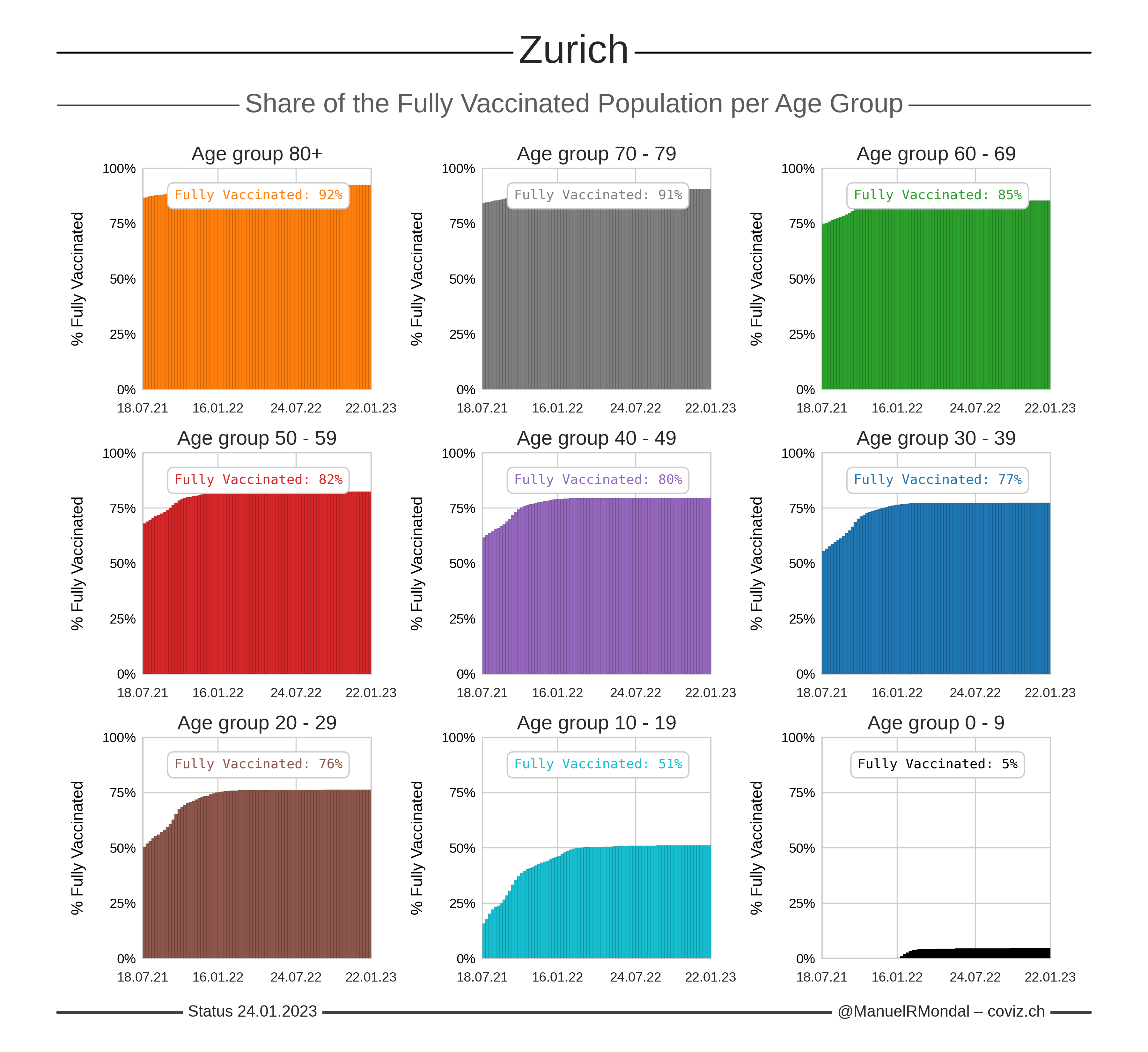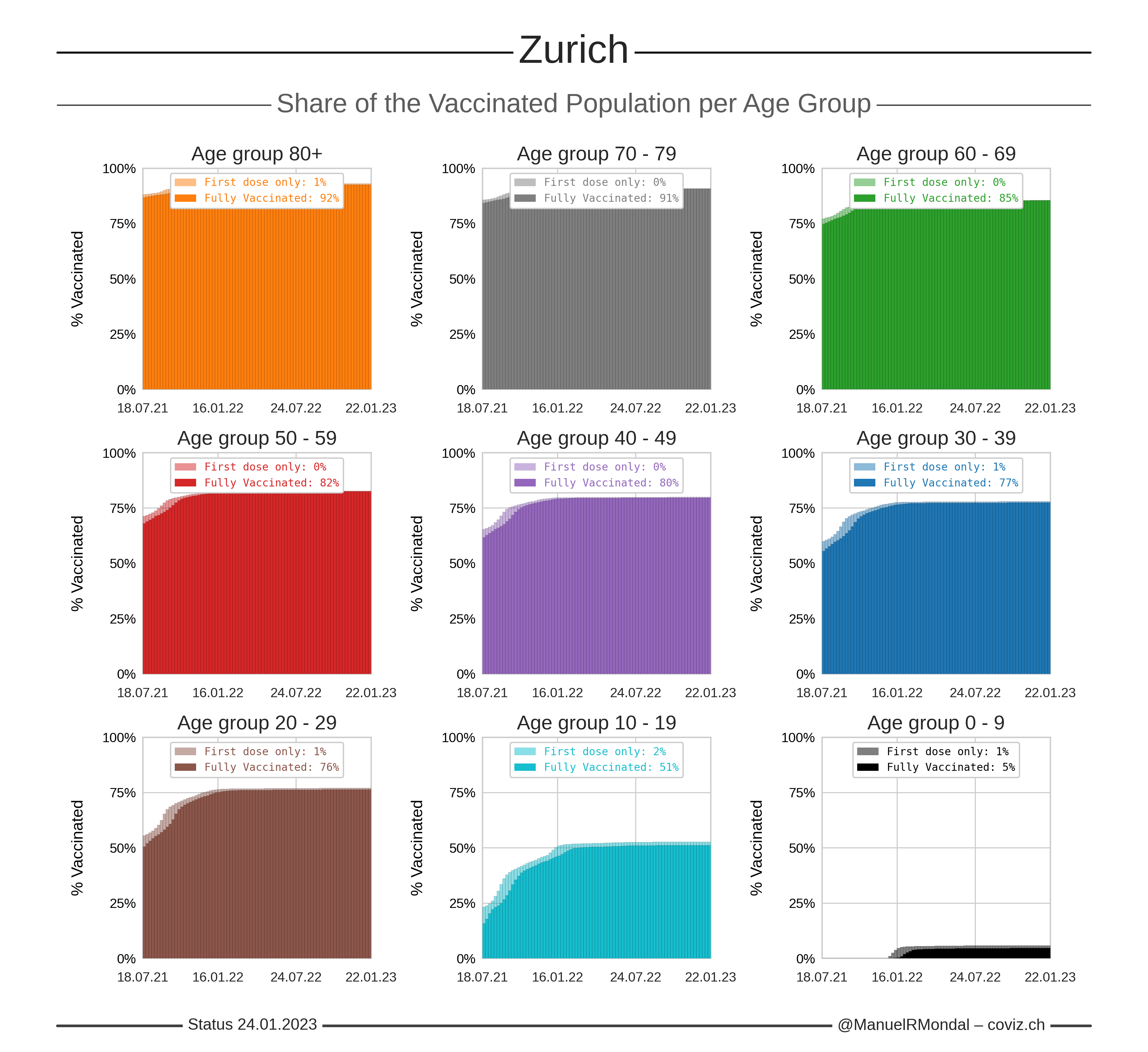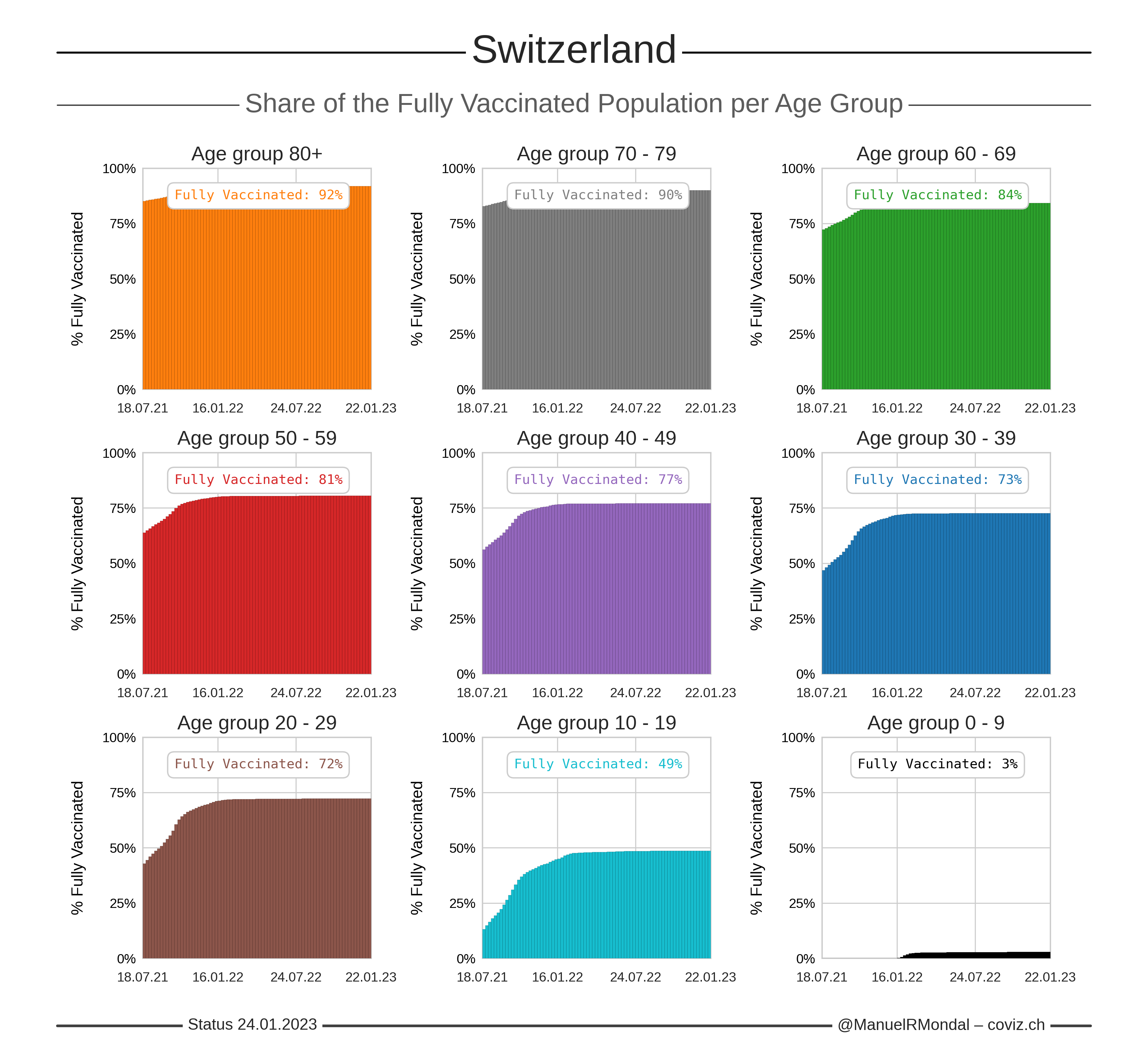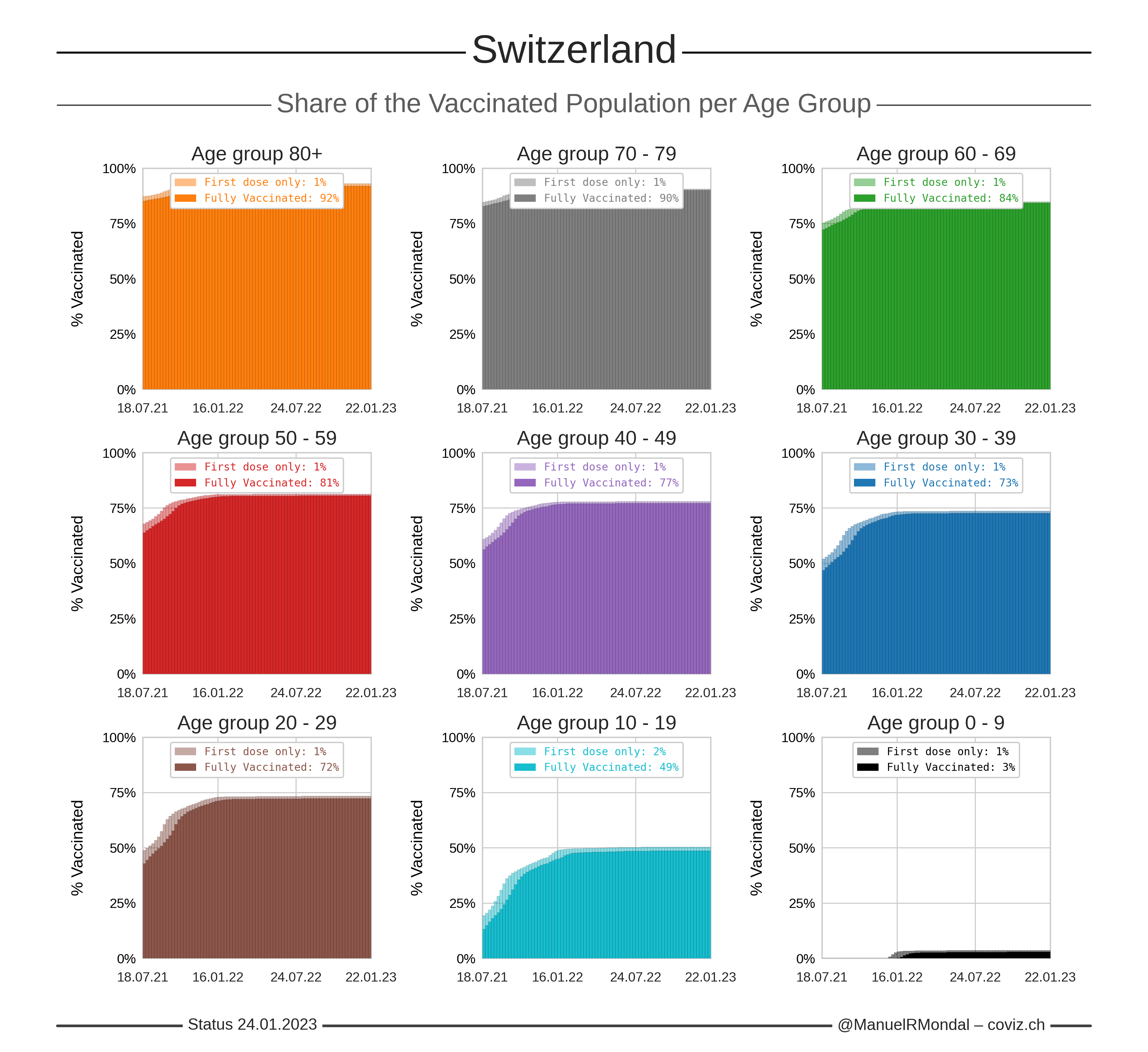Vaccinations by Age Group per Canton
Back to Home 🏠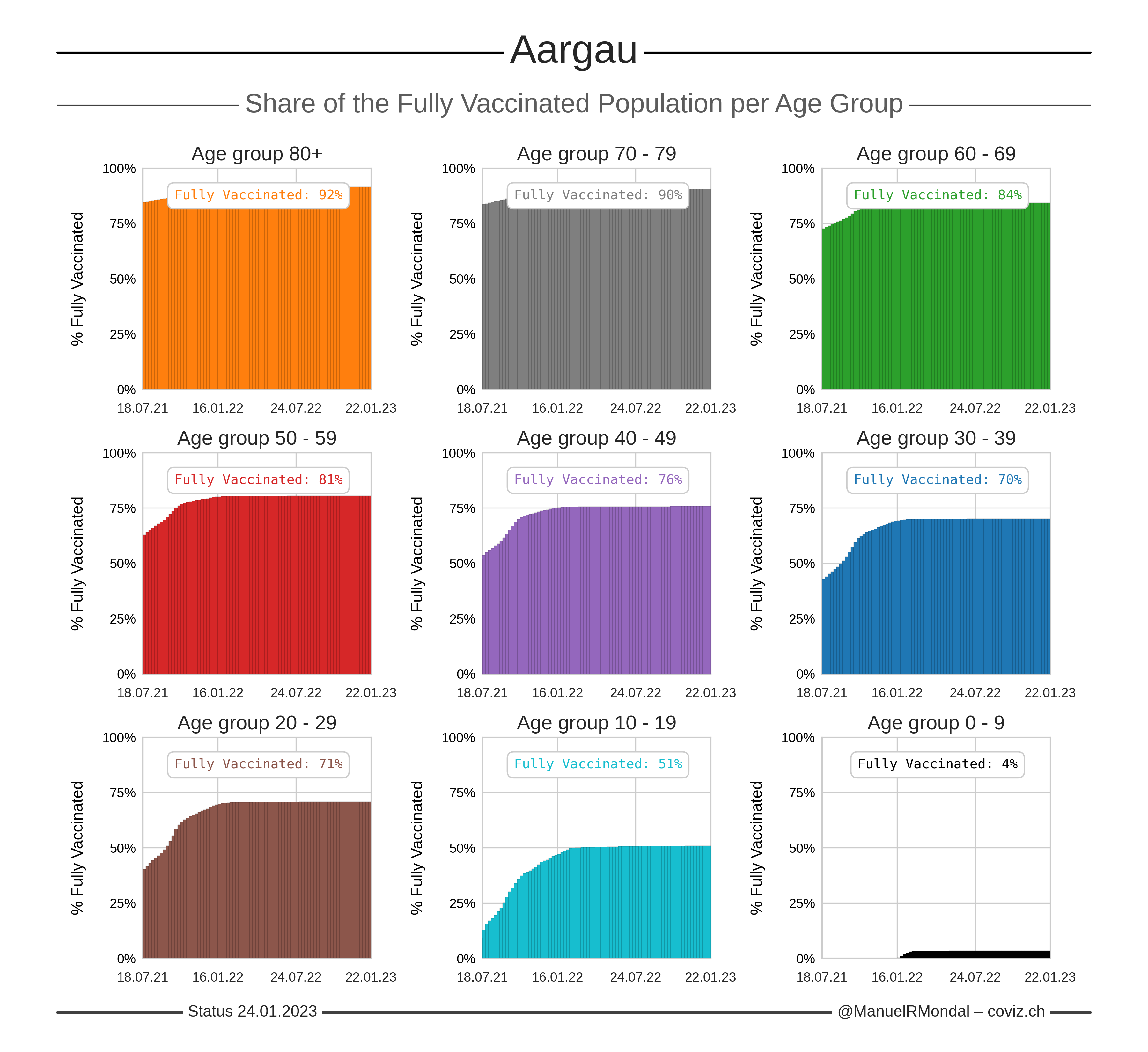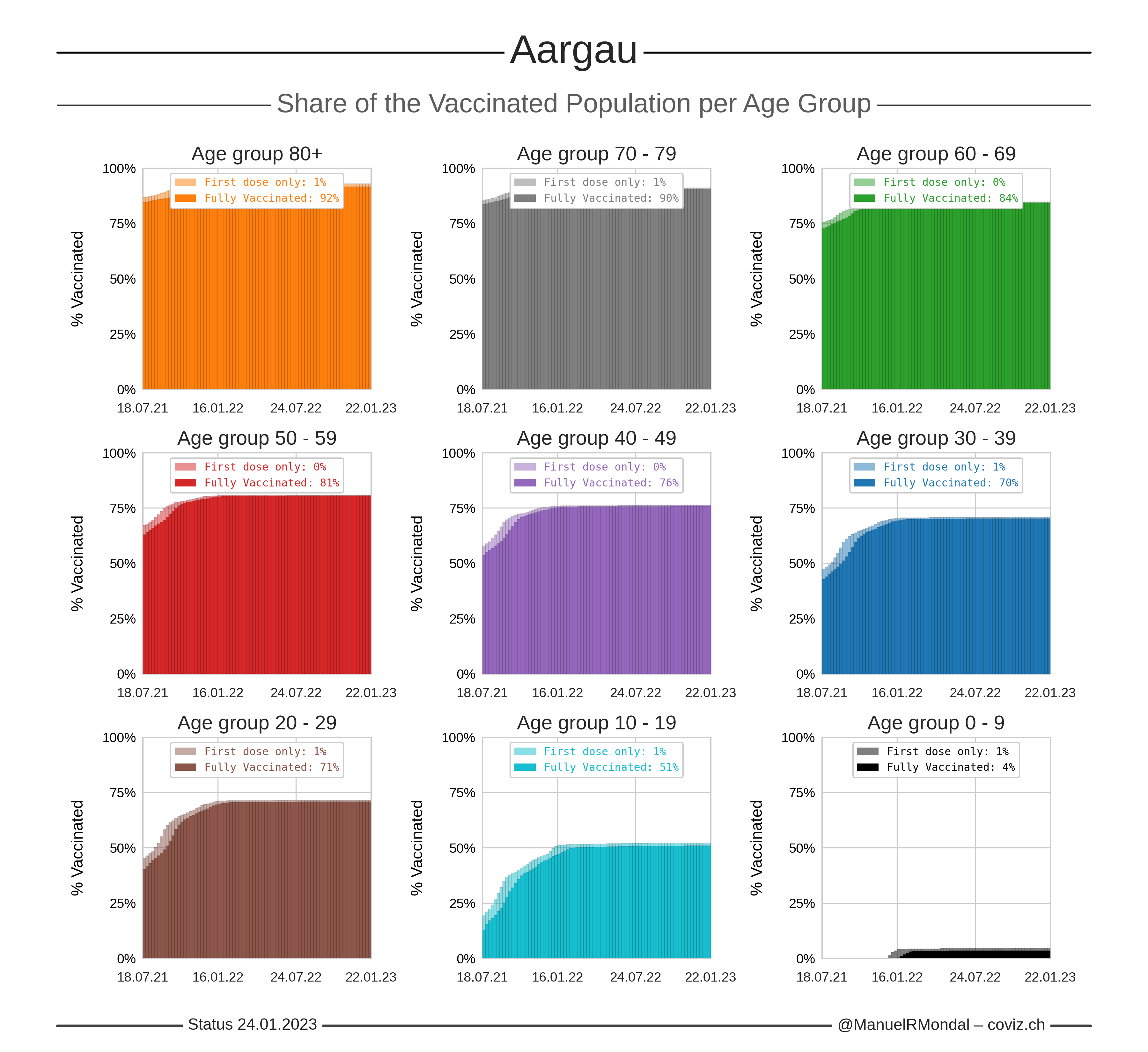Click the image to zoom.
Updated:
Number of first doses calculated from the total number of administered doses and assuming two doses for full vaccinations.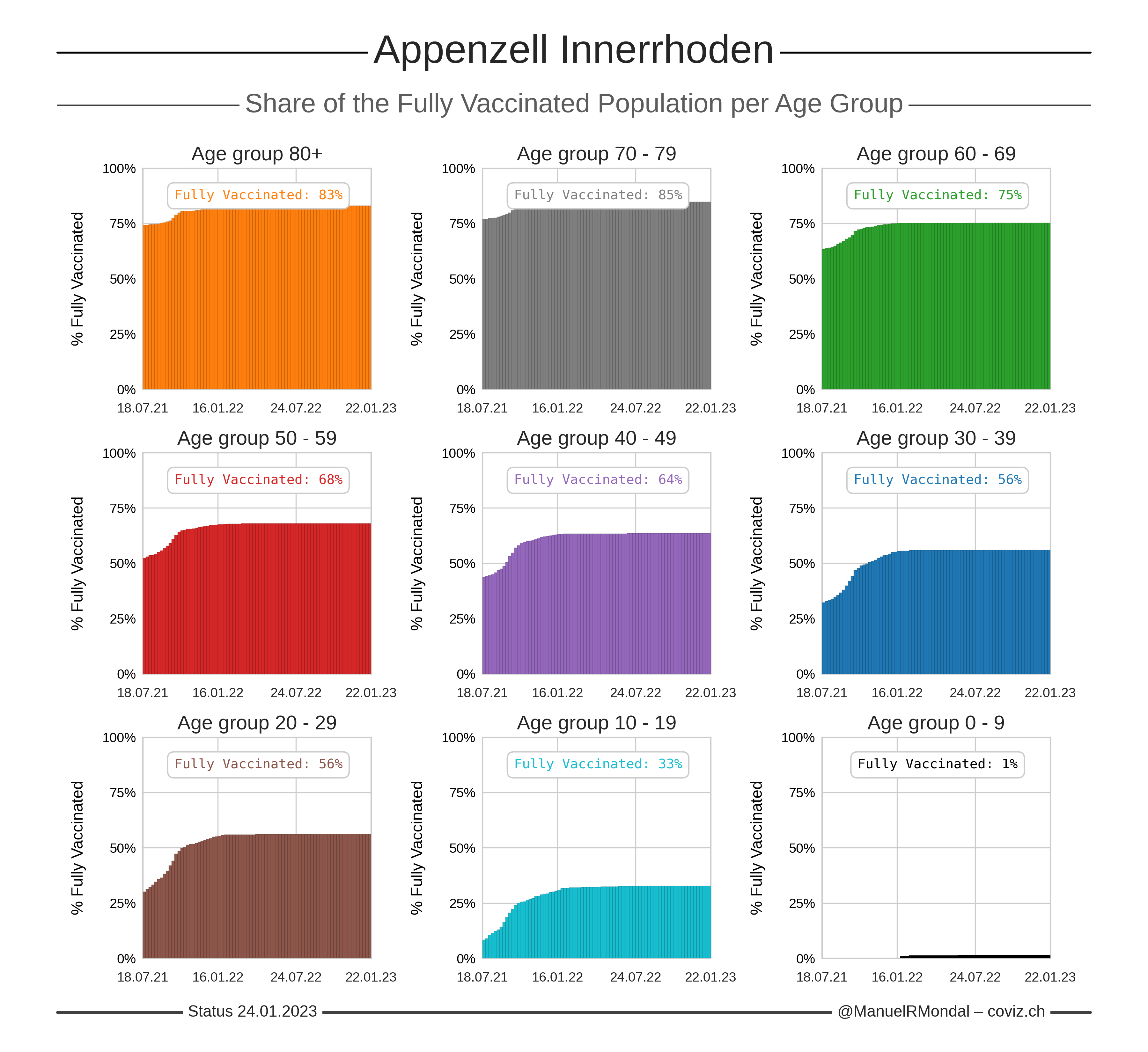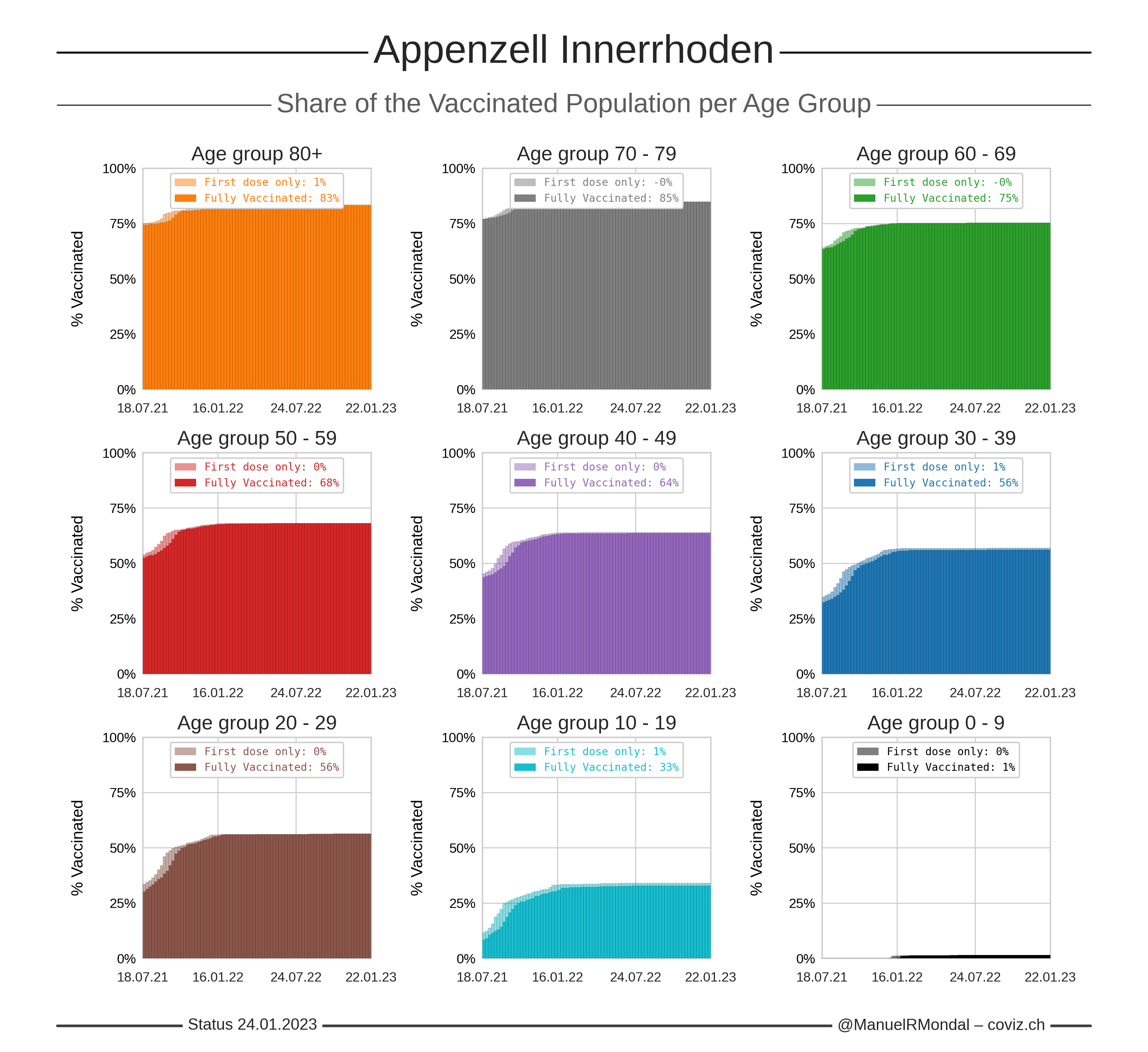Click the image to zoom.
Updated:
Number of first doses calculated from the total number of administered doses and assuming two doses for full vaccinations.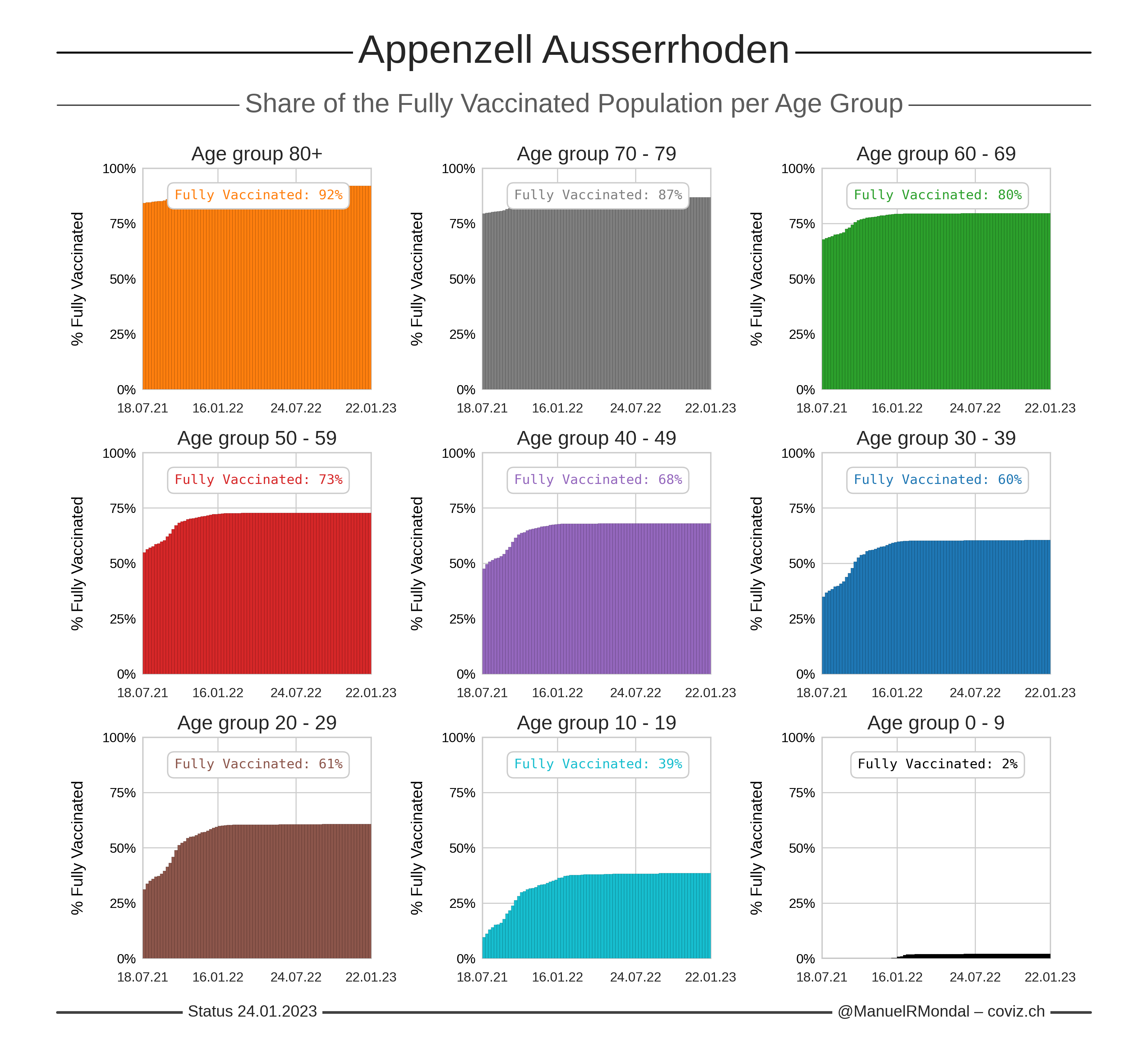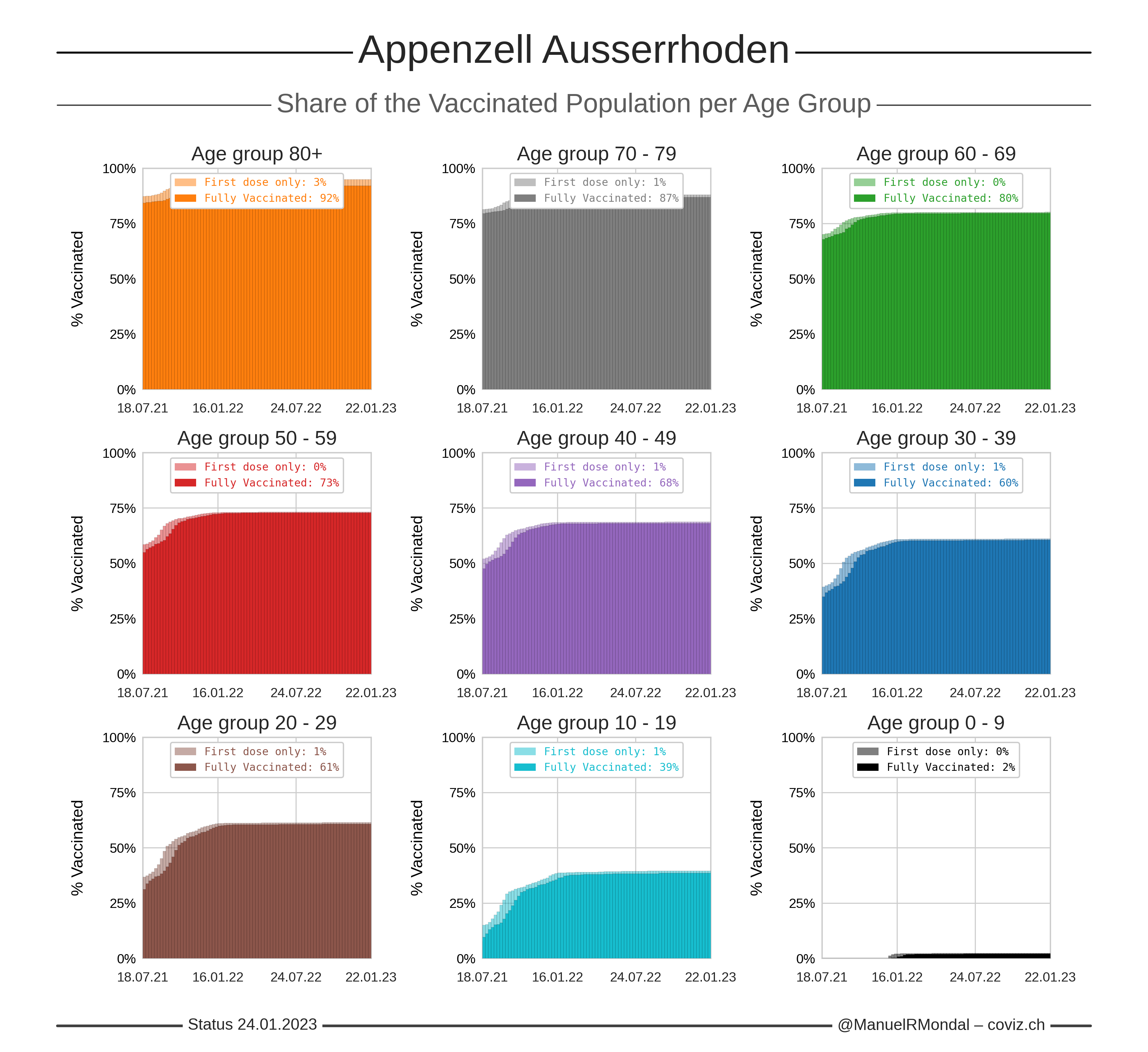Click the image to zoom.
Updated:
Number of first doses calculated from the total number of administered doses and assuming two doses for full vaccinations.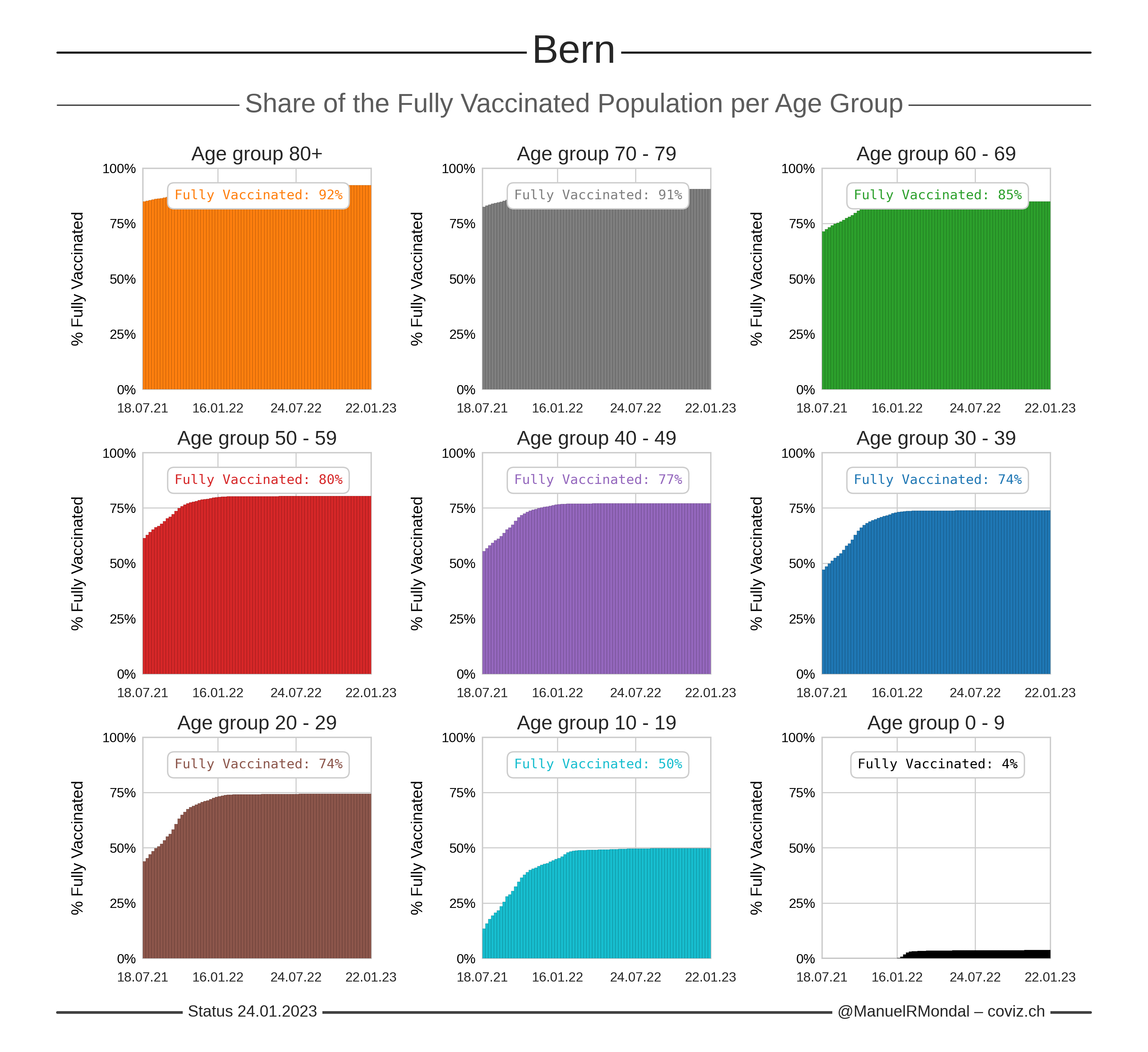Click the image to zoom.
Updated:
Number of first doses calculated from the total number of administered doses and assuming two doses for full vaccinations.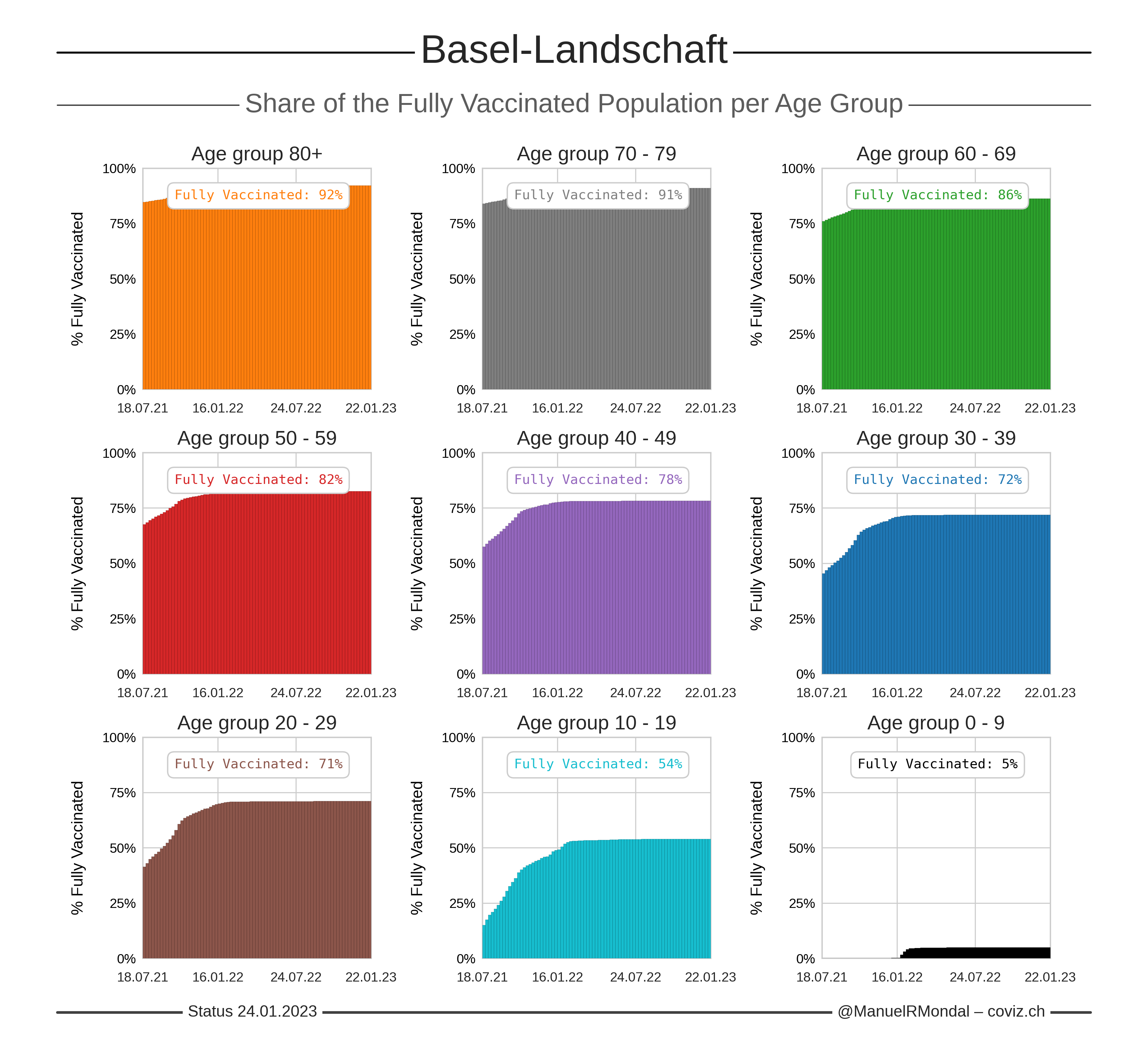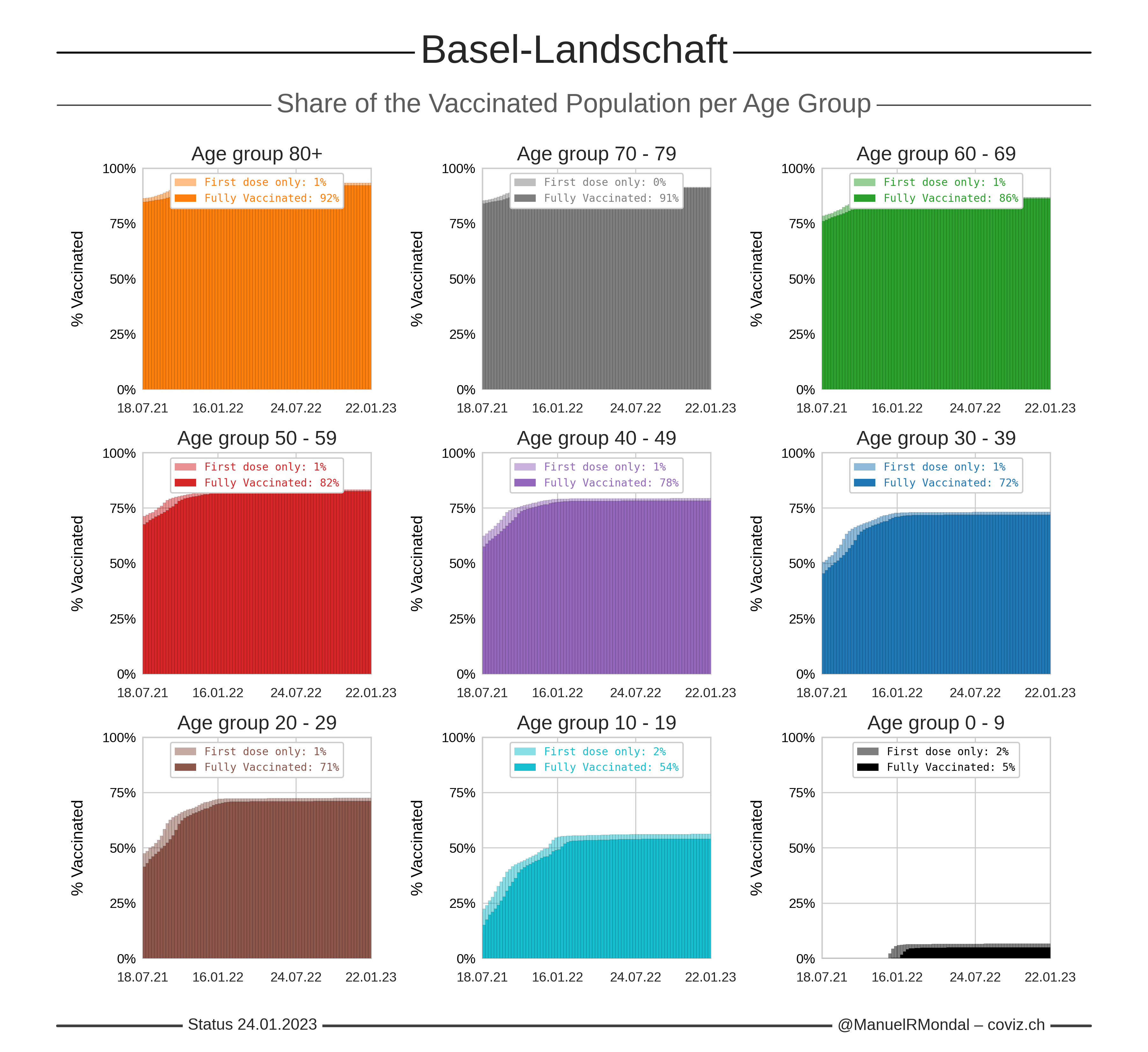Click the image to zoom.
Updated:
Number of first doses calculated from the total number of administered doses and assuming two doses for full vaccinations.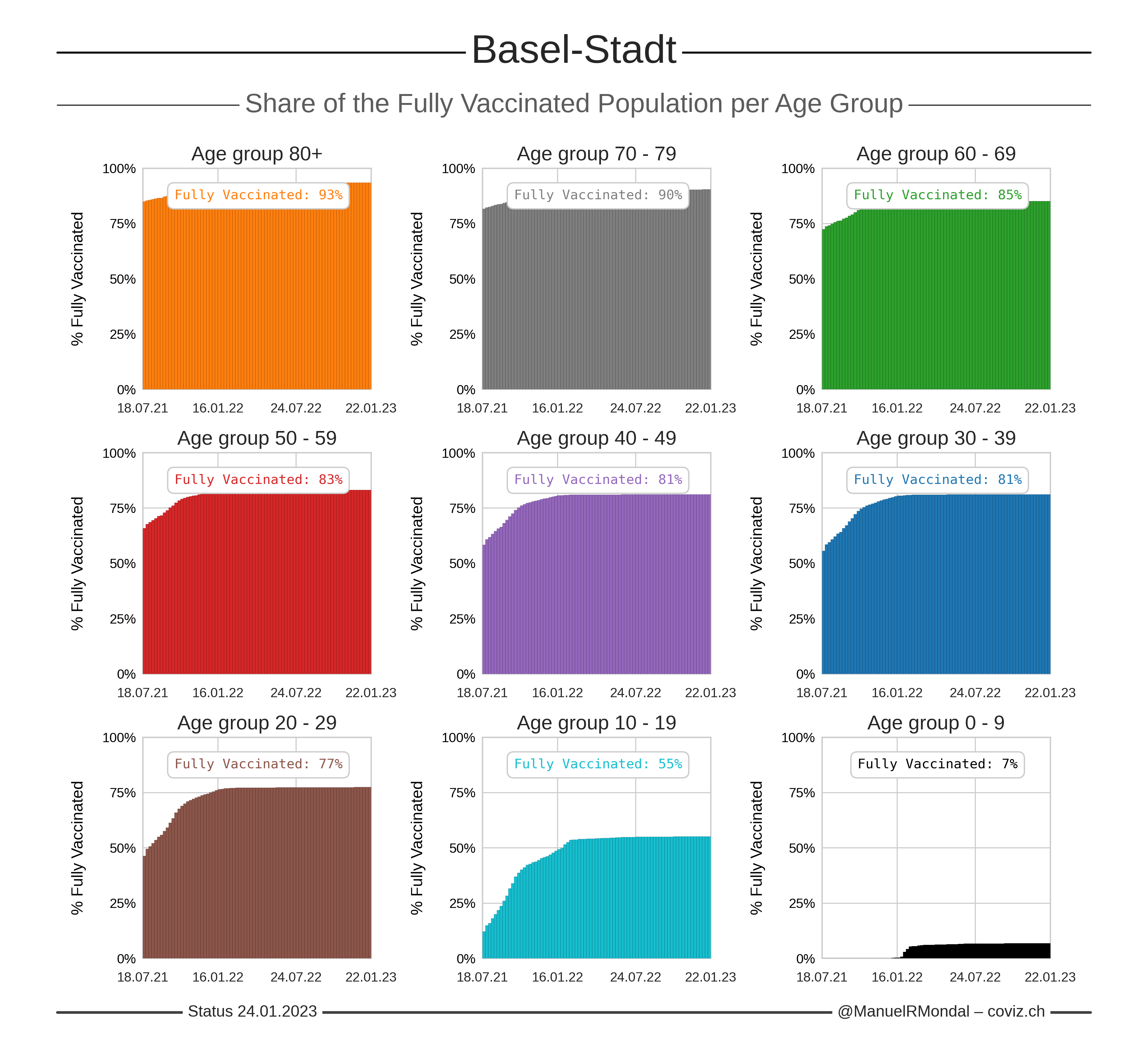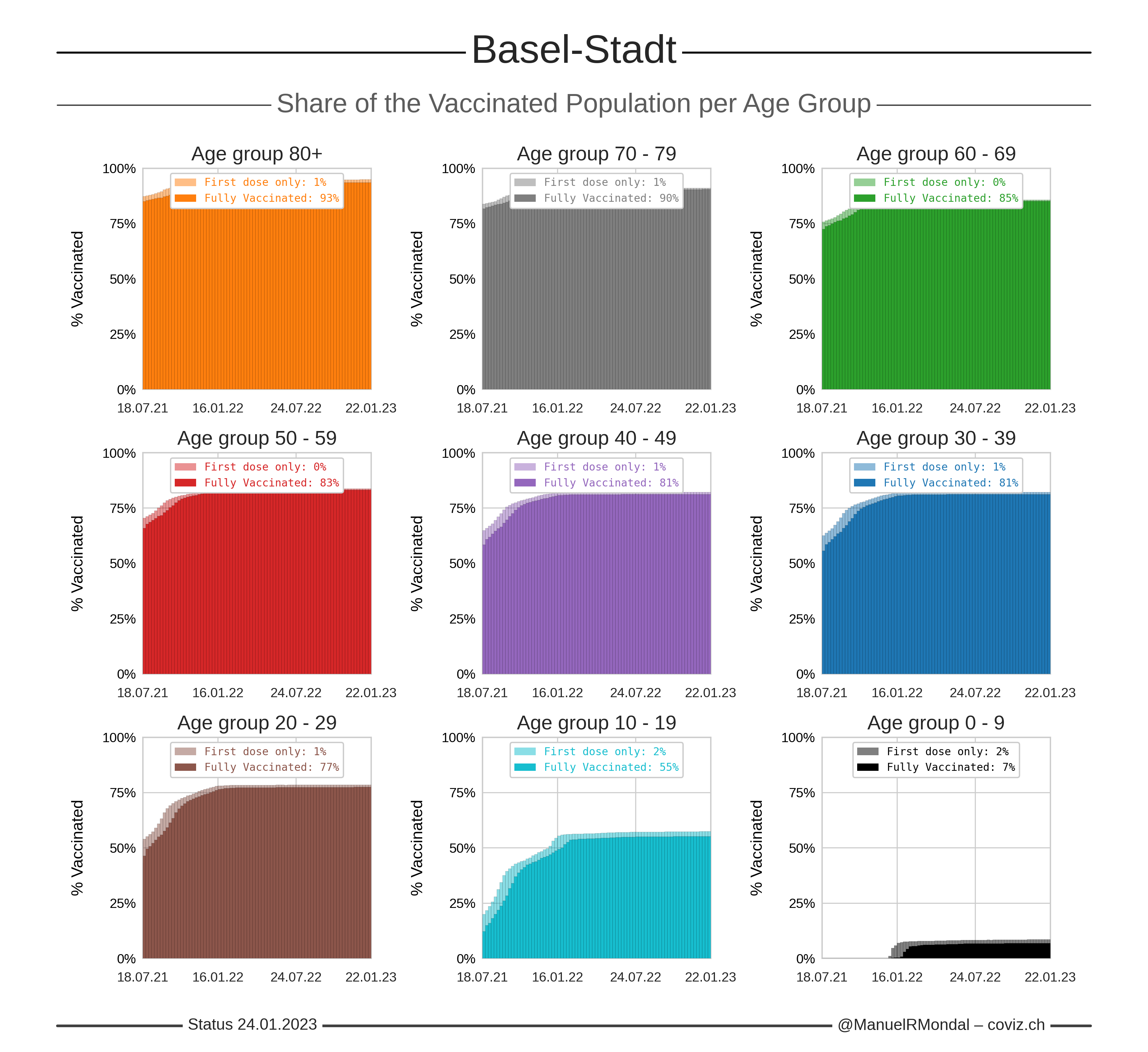Click the image to zoom.
Updated:
Number of first doses calculated from the total number of administered doses and assuming two doses for full vaccinations.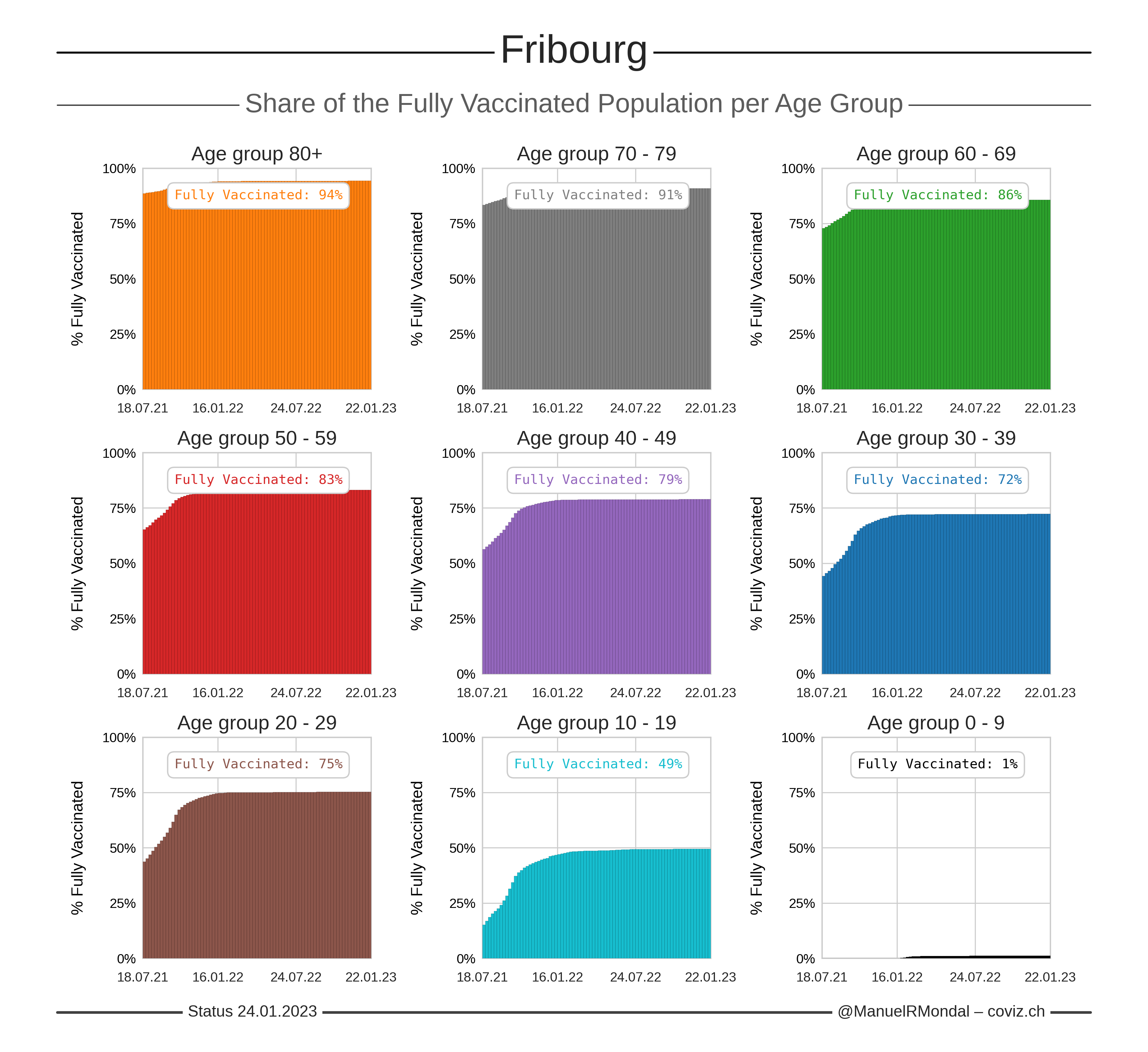Click the image to zoom.
Updated:
Number of first doses calculated from the total number of administered doses and assuming two doses for full vaccinations.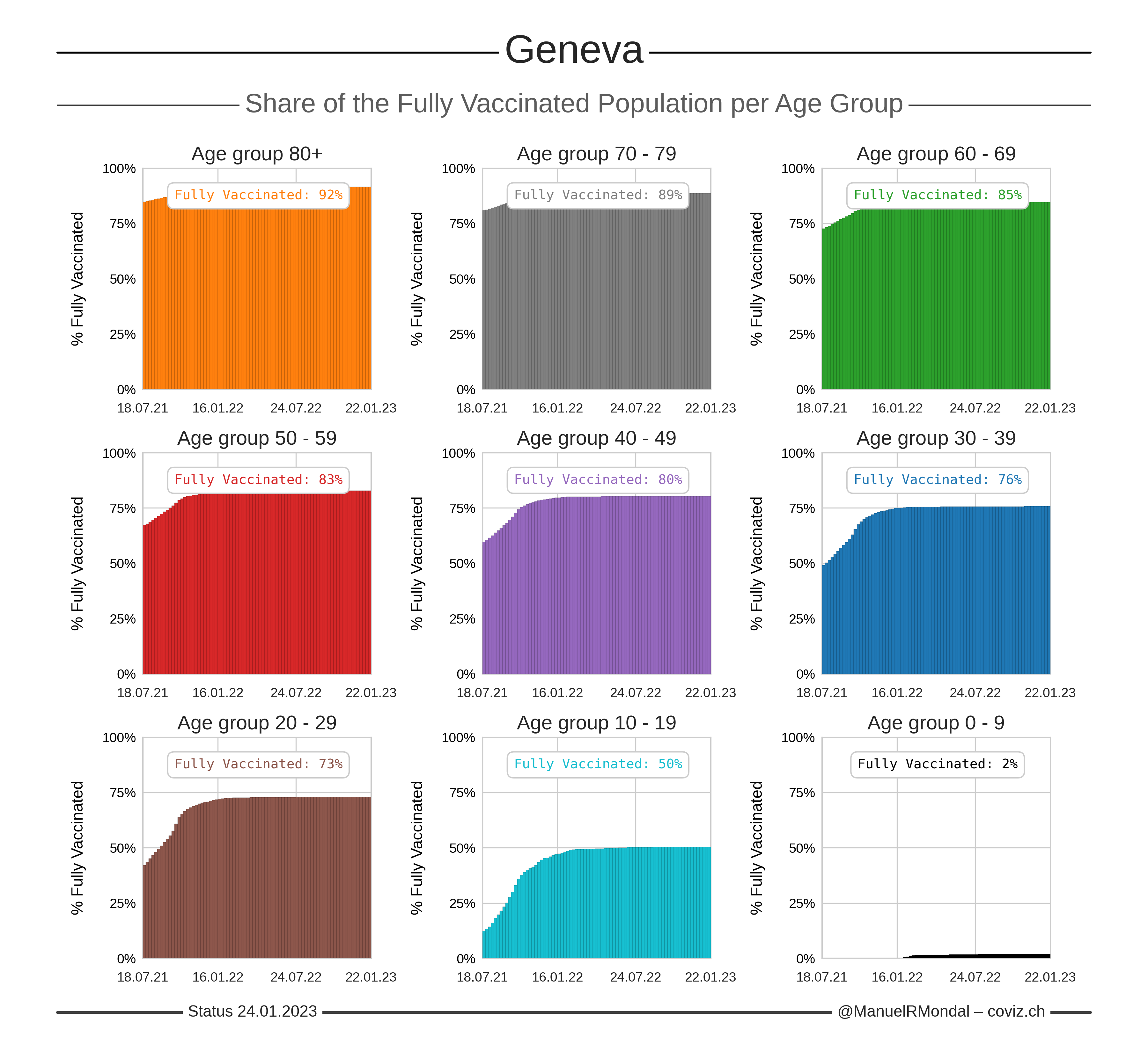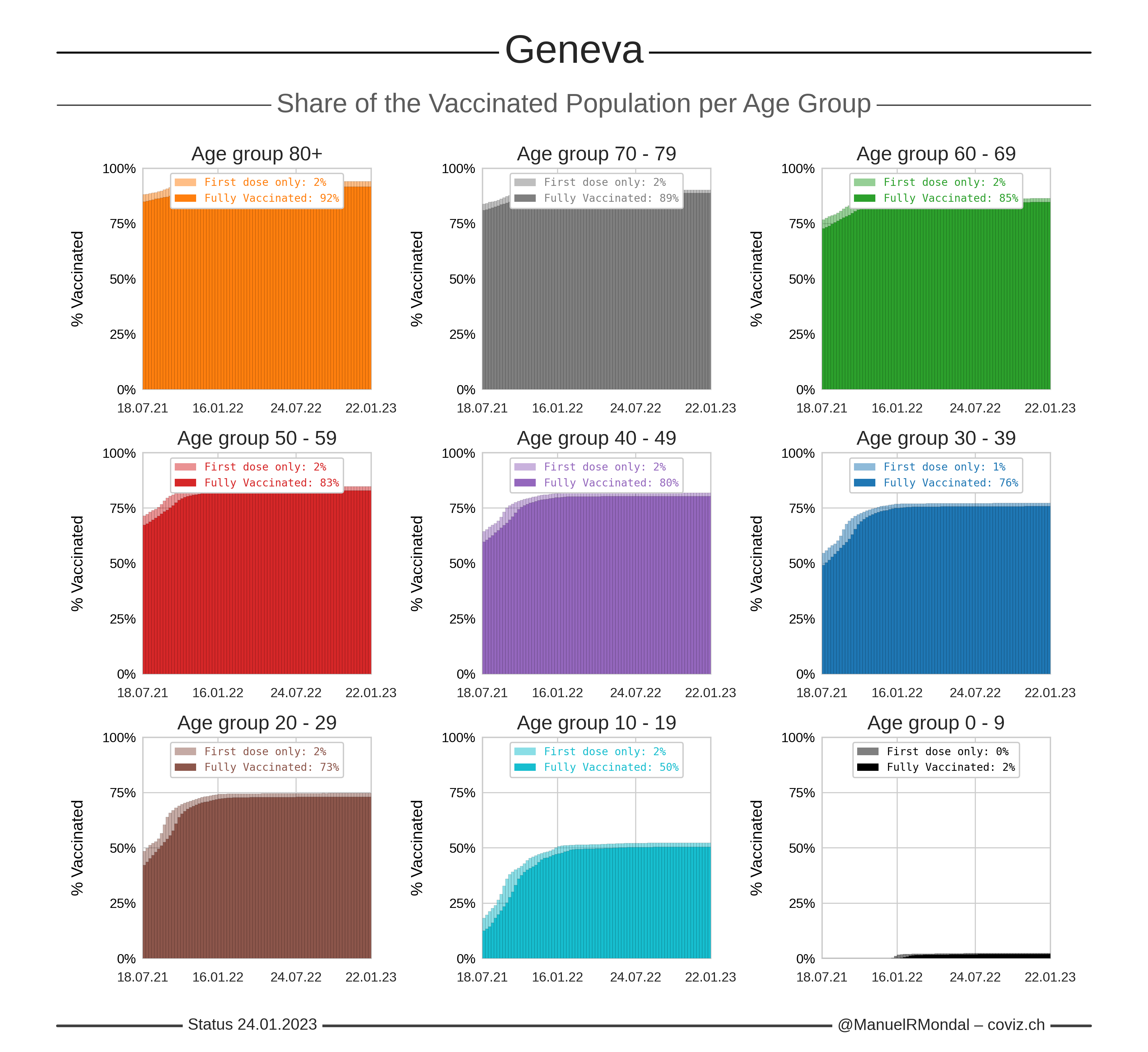Click the image to zoom.
Updated:
Number of first doses calculated from the total number of administered doses and assuming two doses for full vaccinations.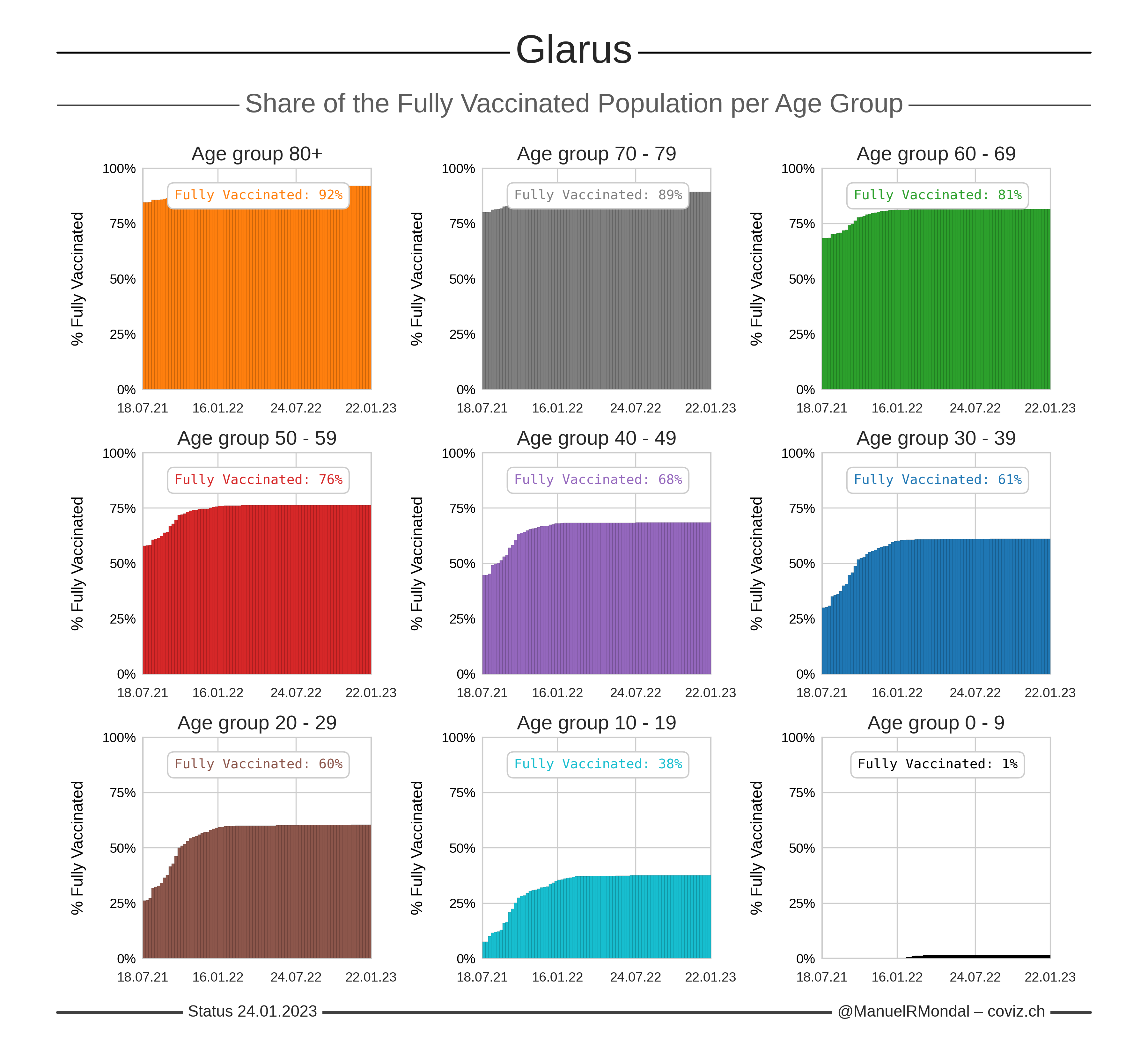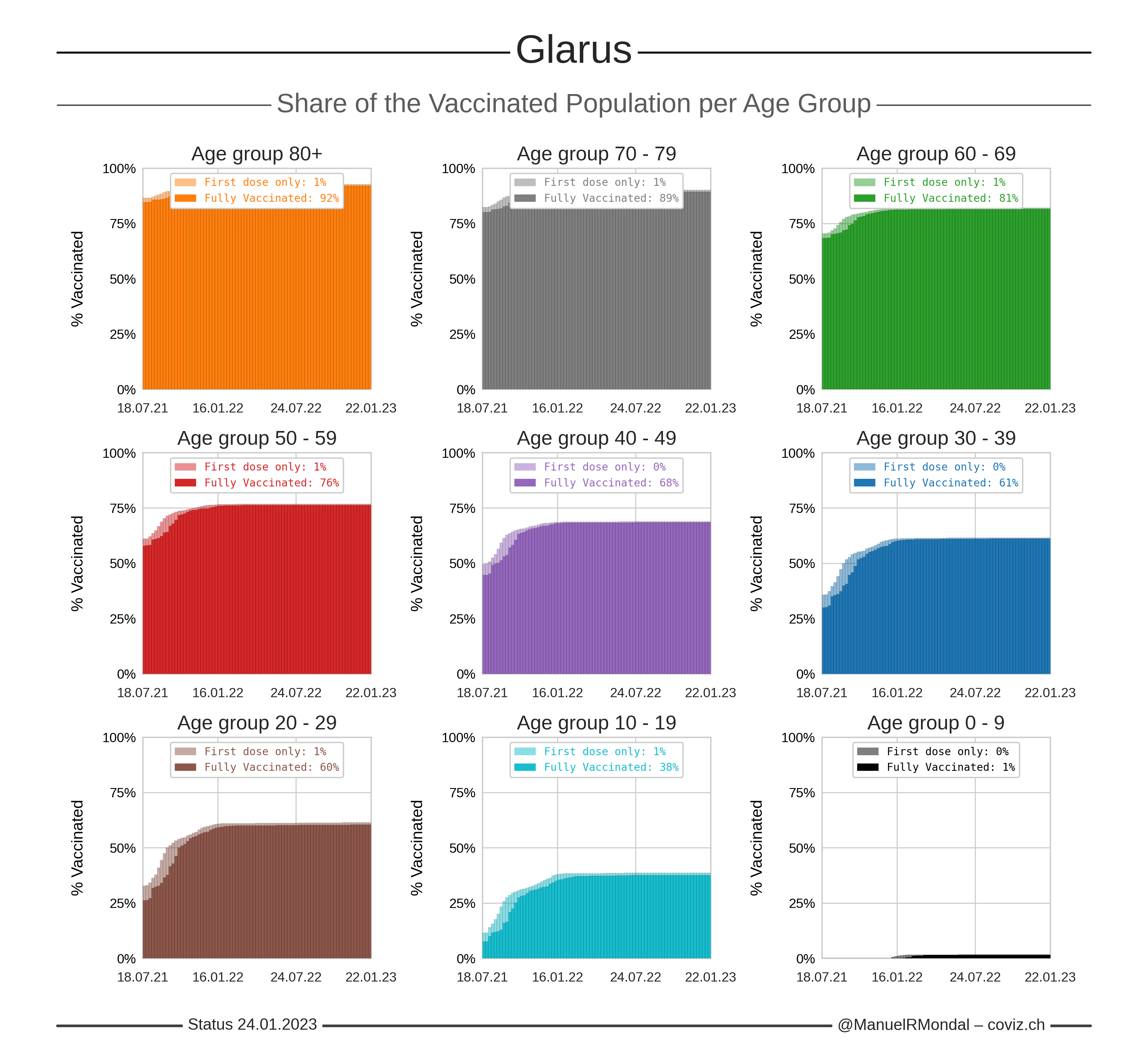Click the image to zoom.
Updated:
Number of first doses calculated from the total number of administered doses and assuming two doses for full vaccinations.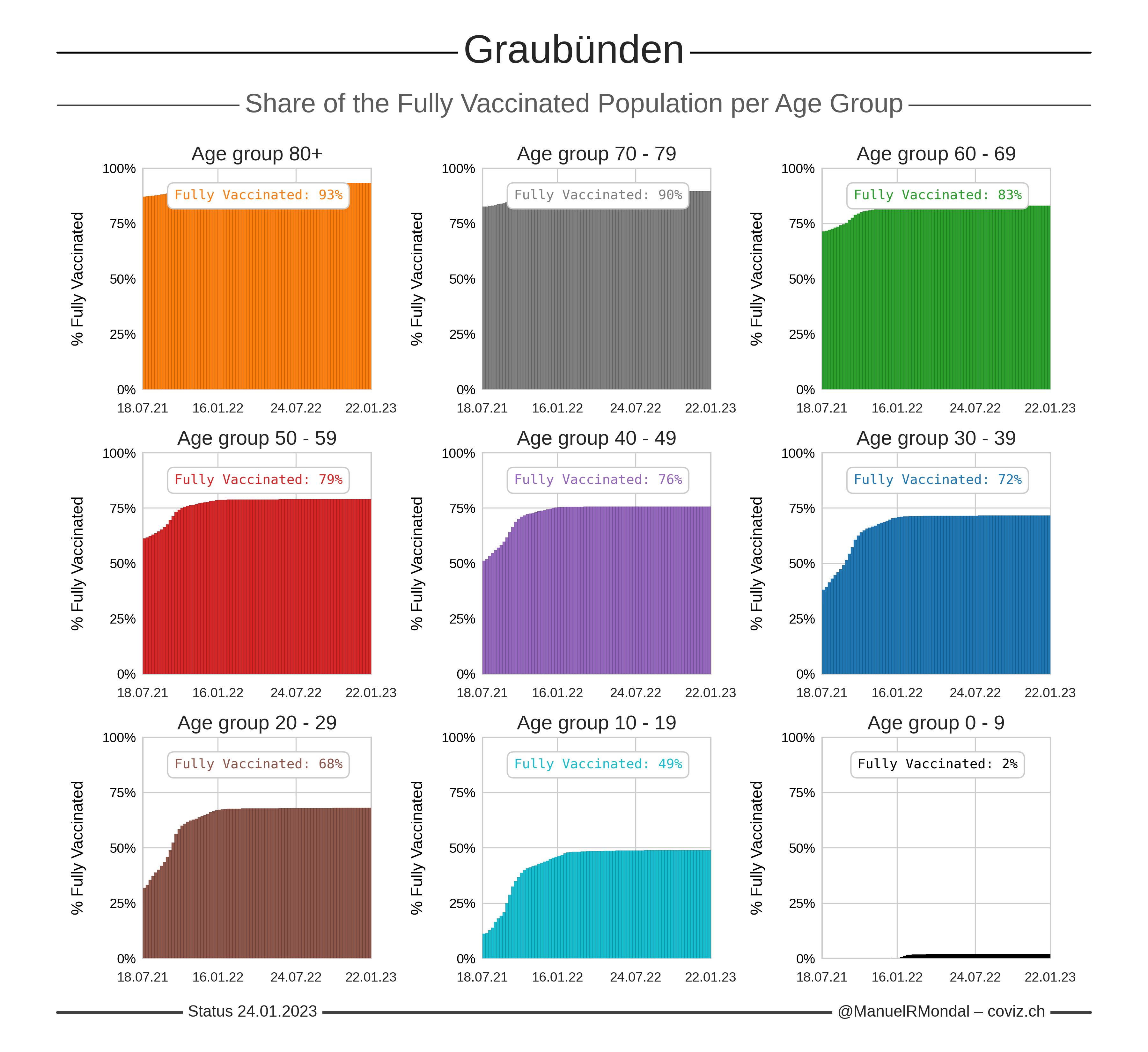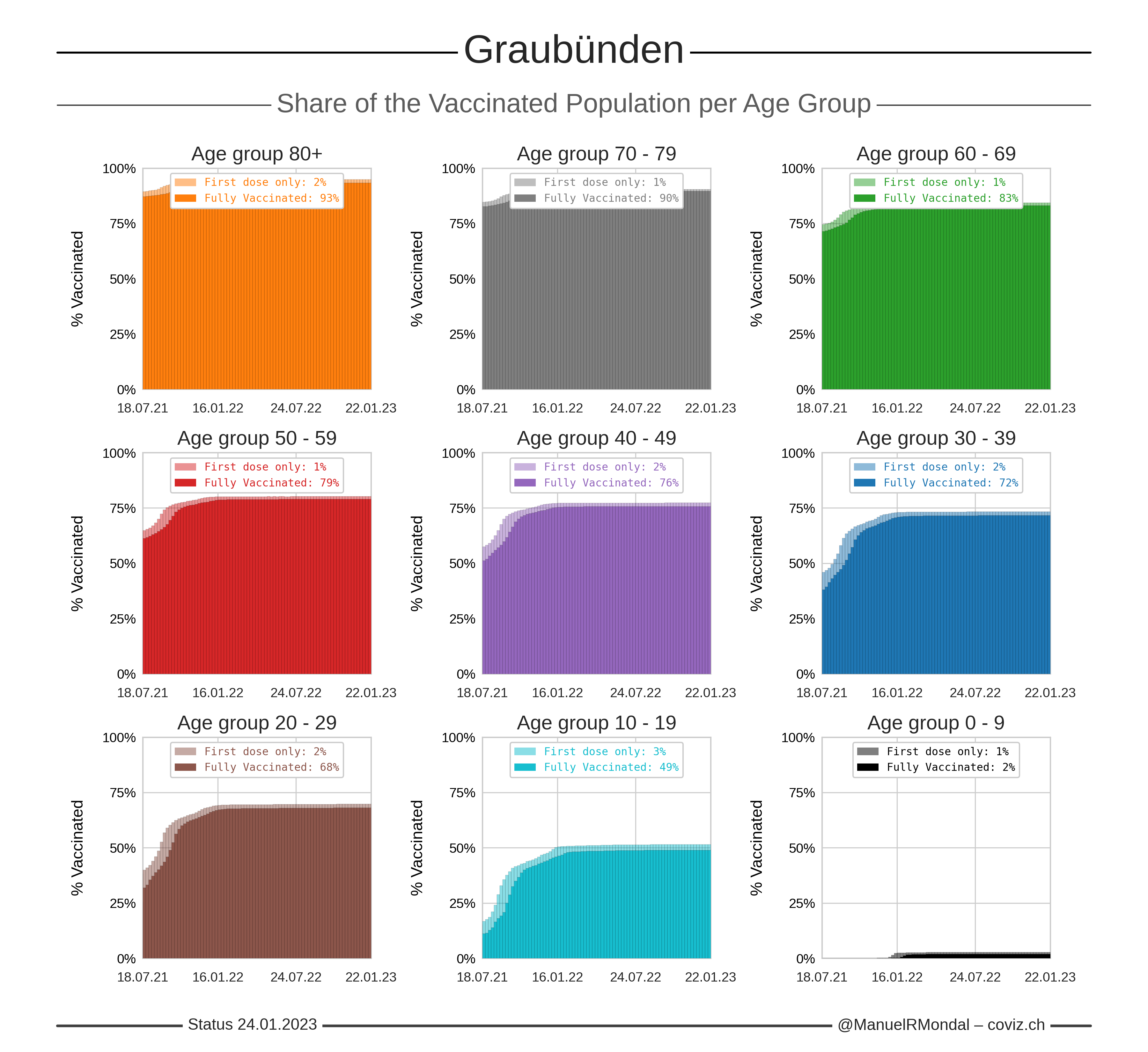Click the image to zoom.
Updated:
Number of first doses calculated from the total number of administered doses and assuming two doses for full vaccinations.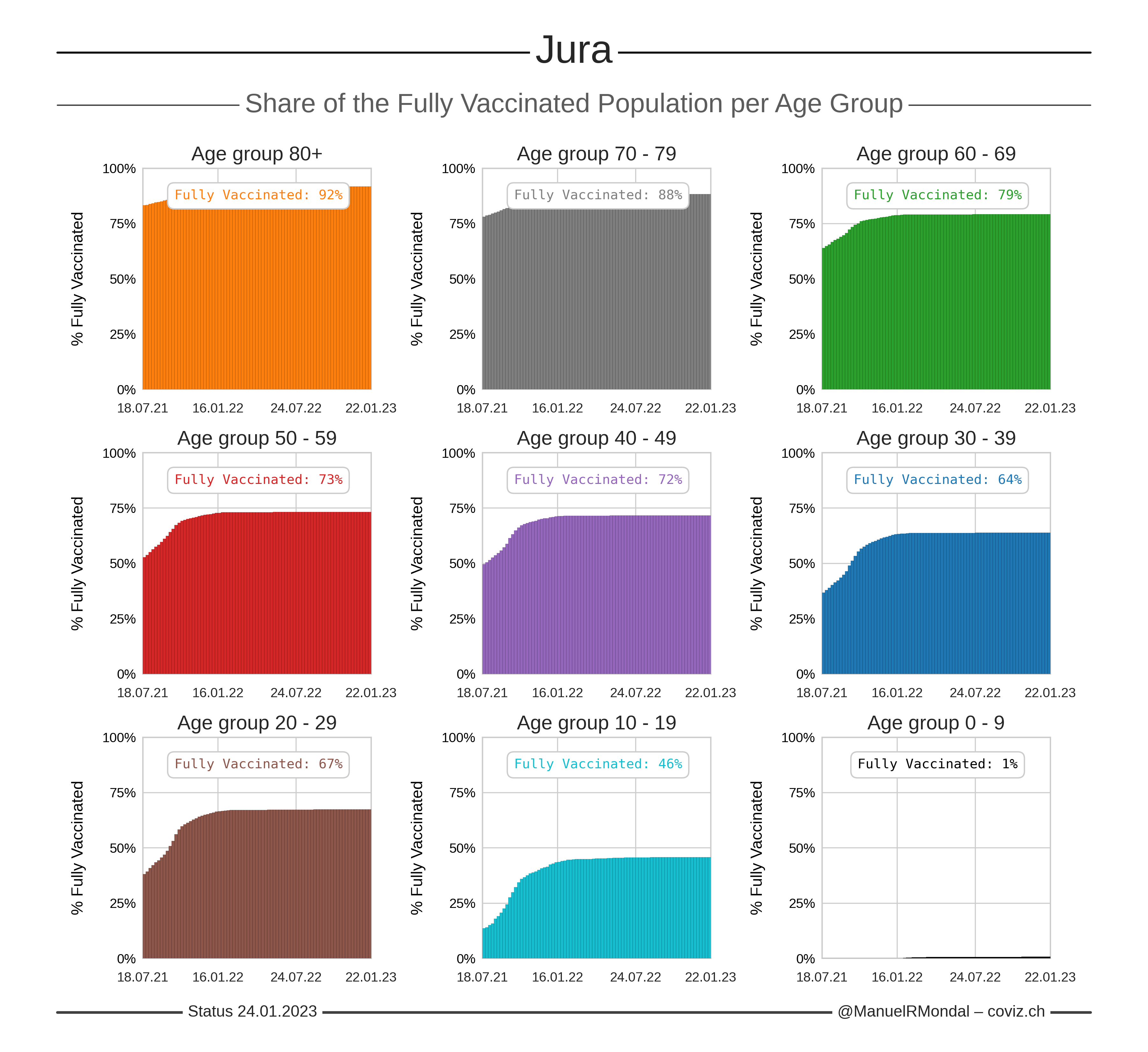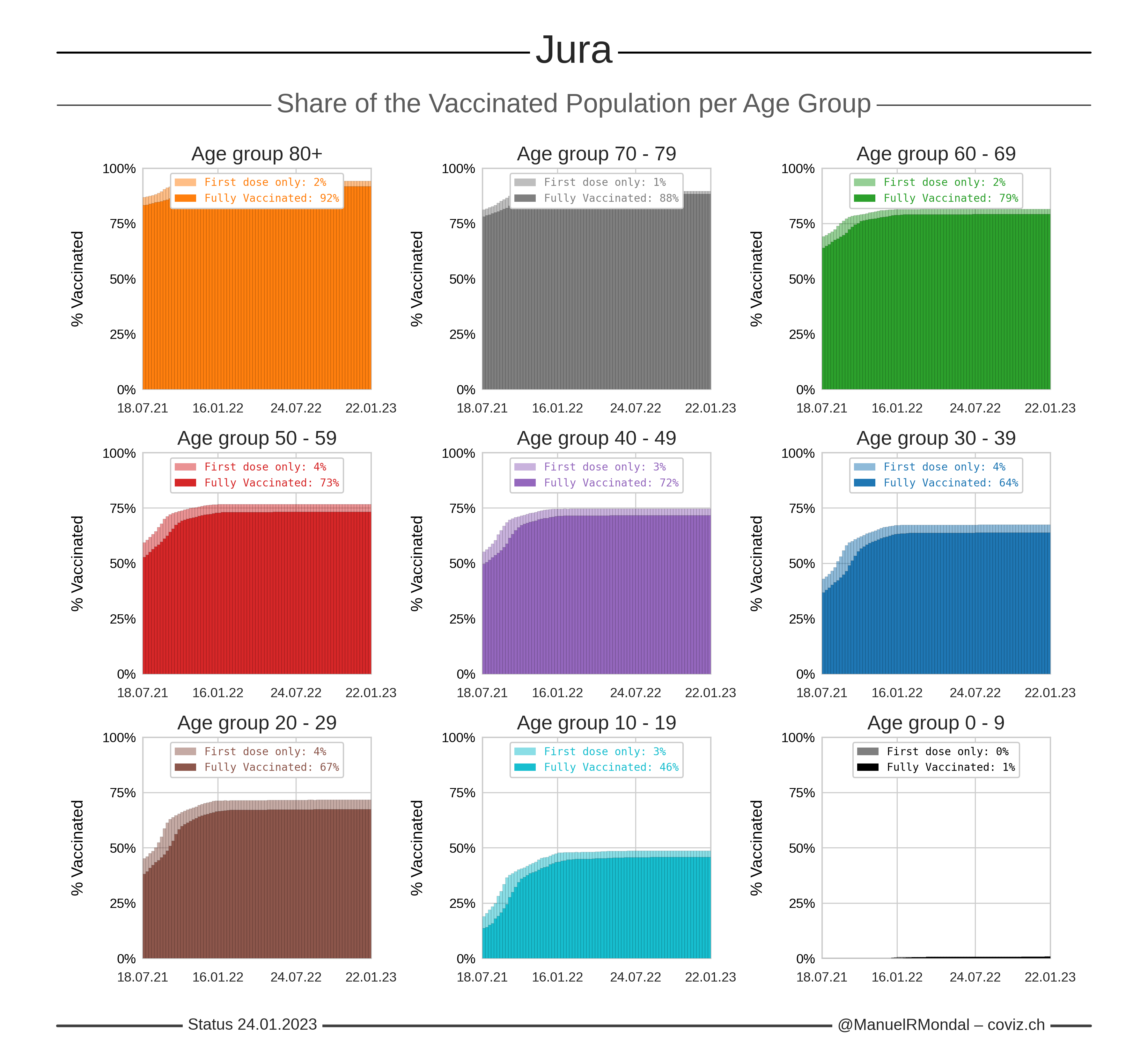Click the image to zoom.
Updated:
Number of first doses calculated from the total number of administered doses and assuming two doses for full vaccinations.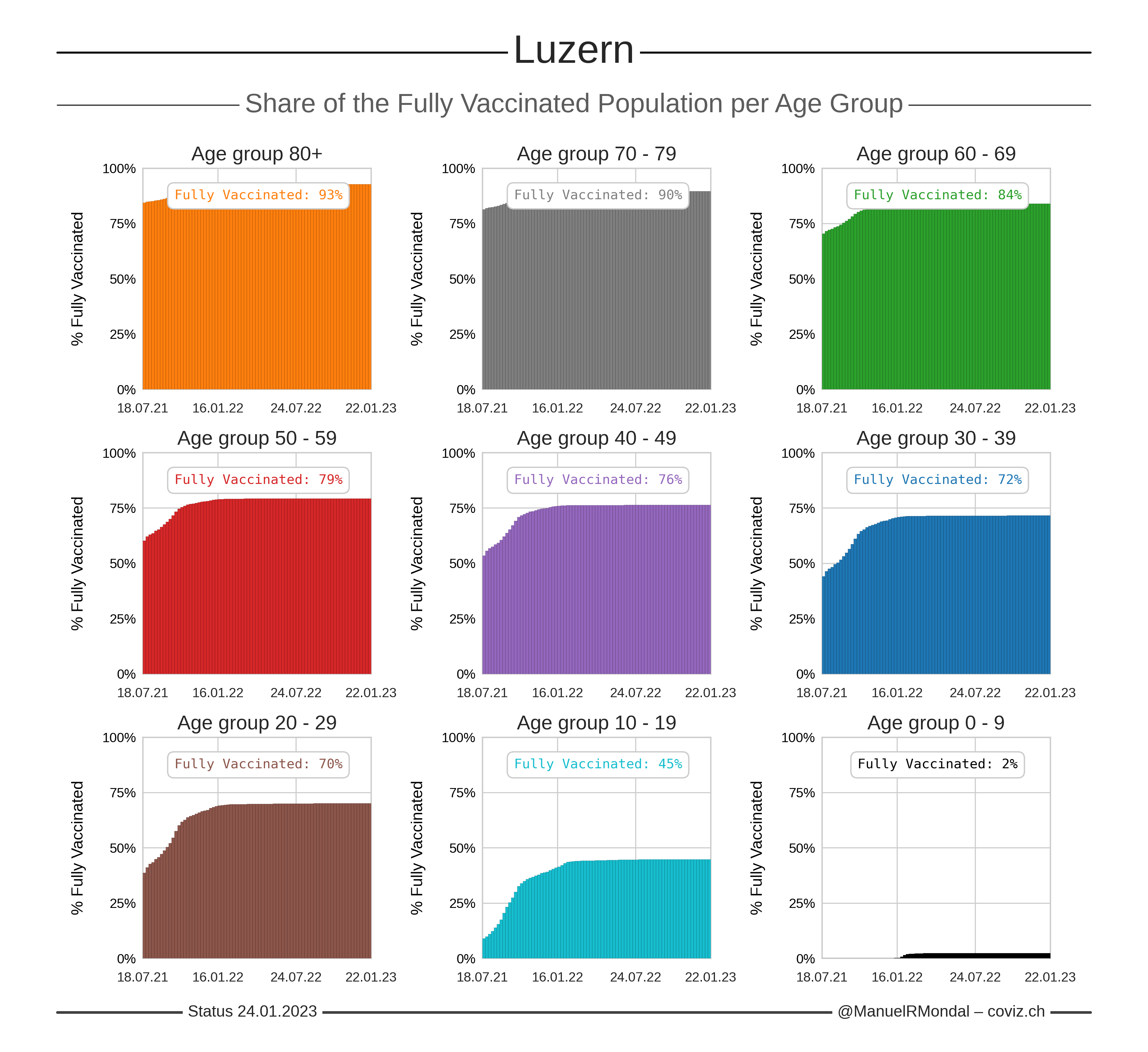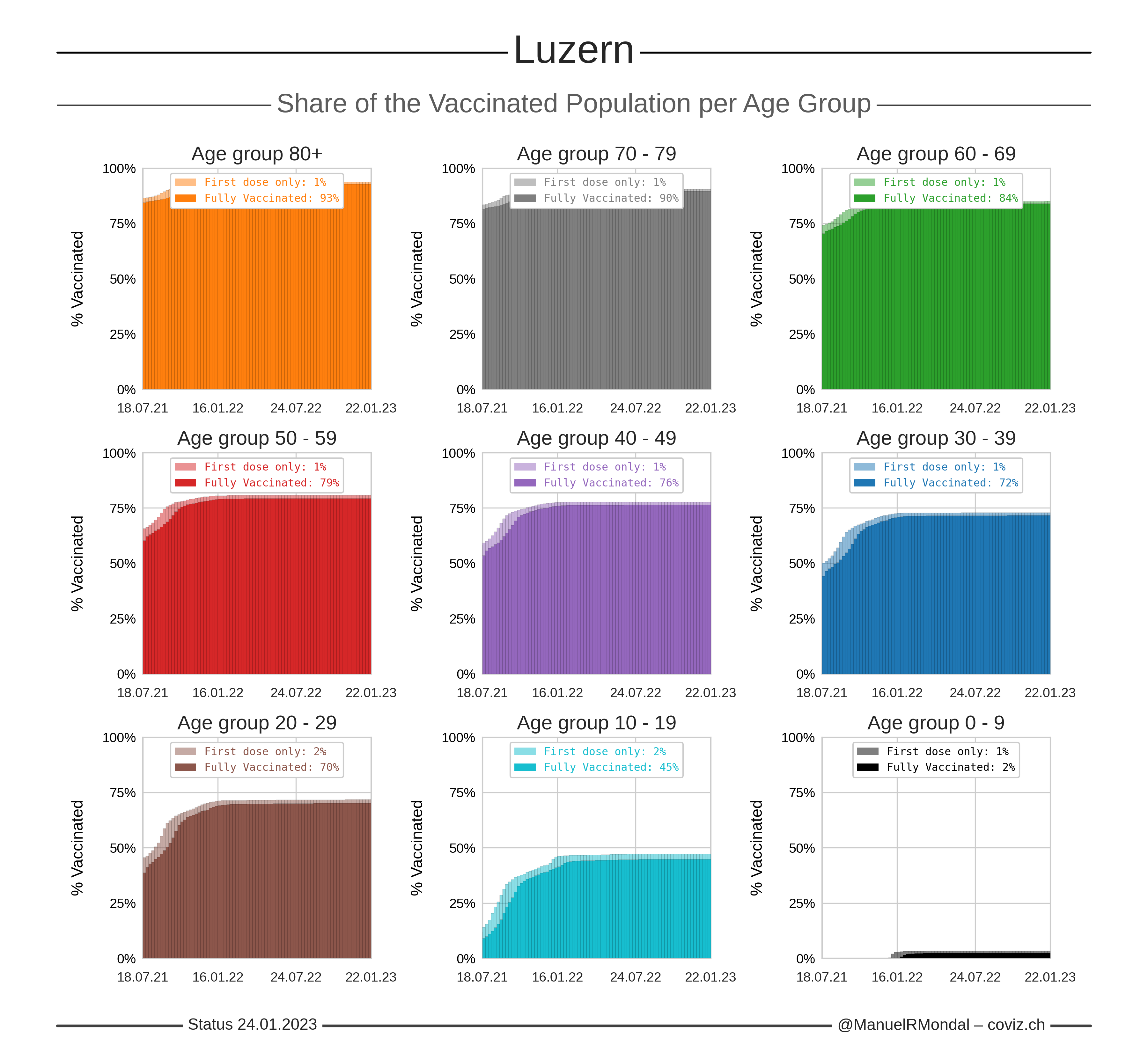Click the image to zoom.
Updated:
Number of first doses calculated from the total number of administered doses and assuming two doses for full vaccinations.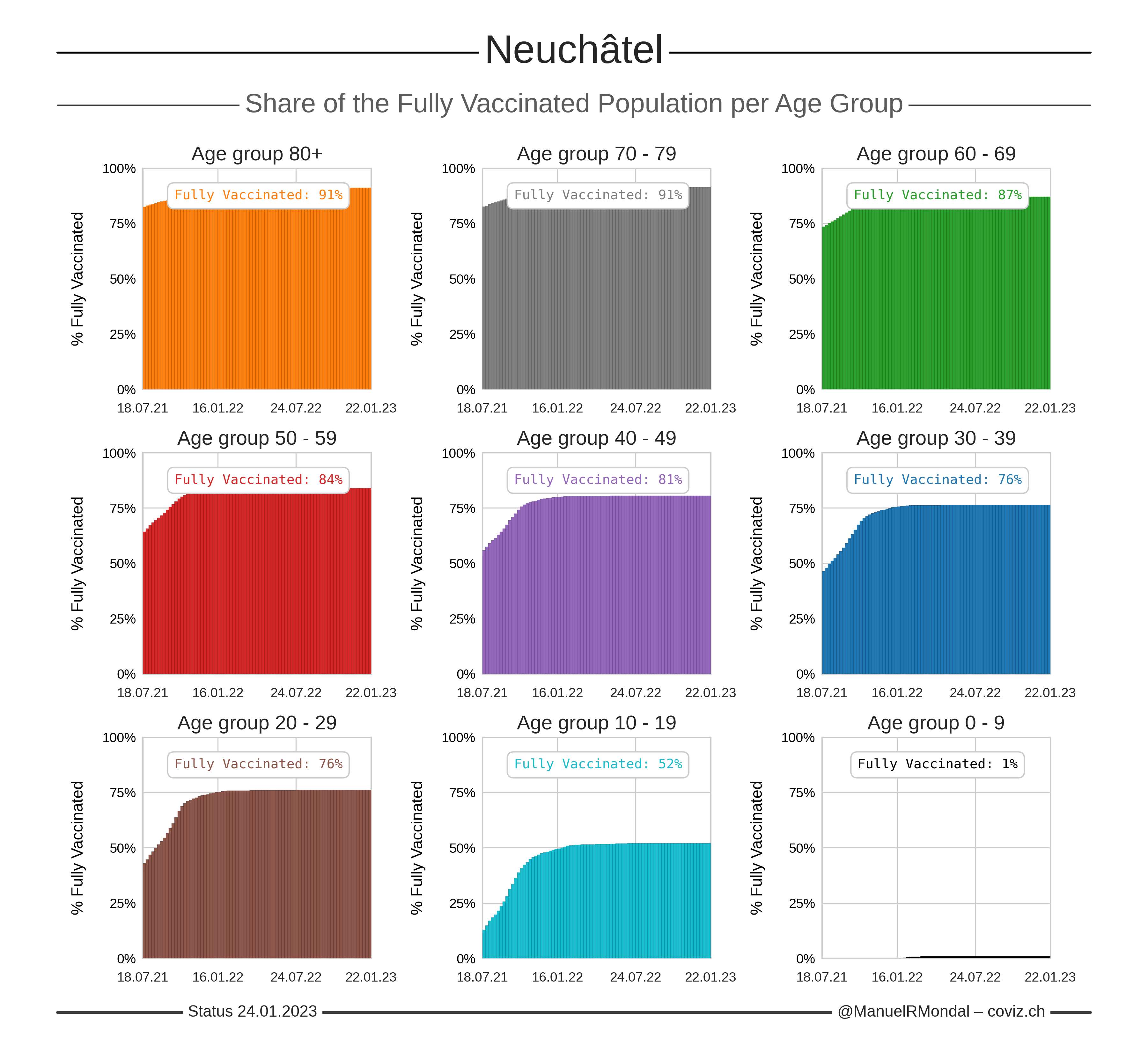Click the image to zoom.
Updated:
Number of first doses calculated from the total number of administered doses and assuming two doses for full vaccinations.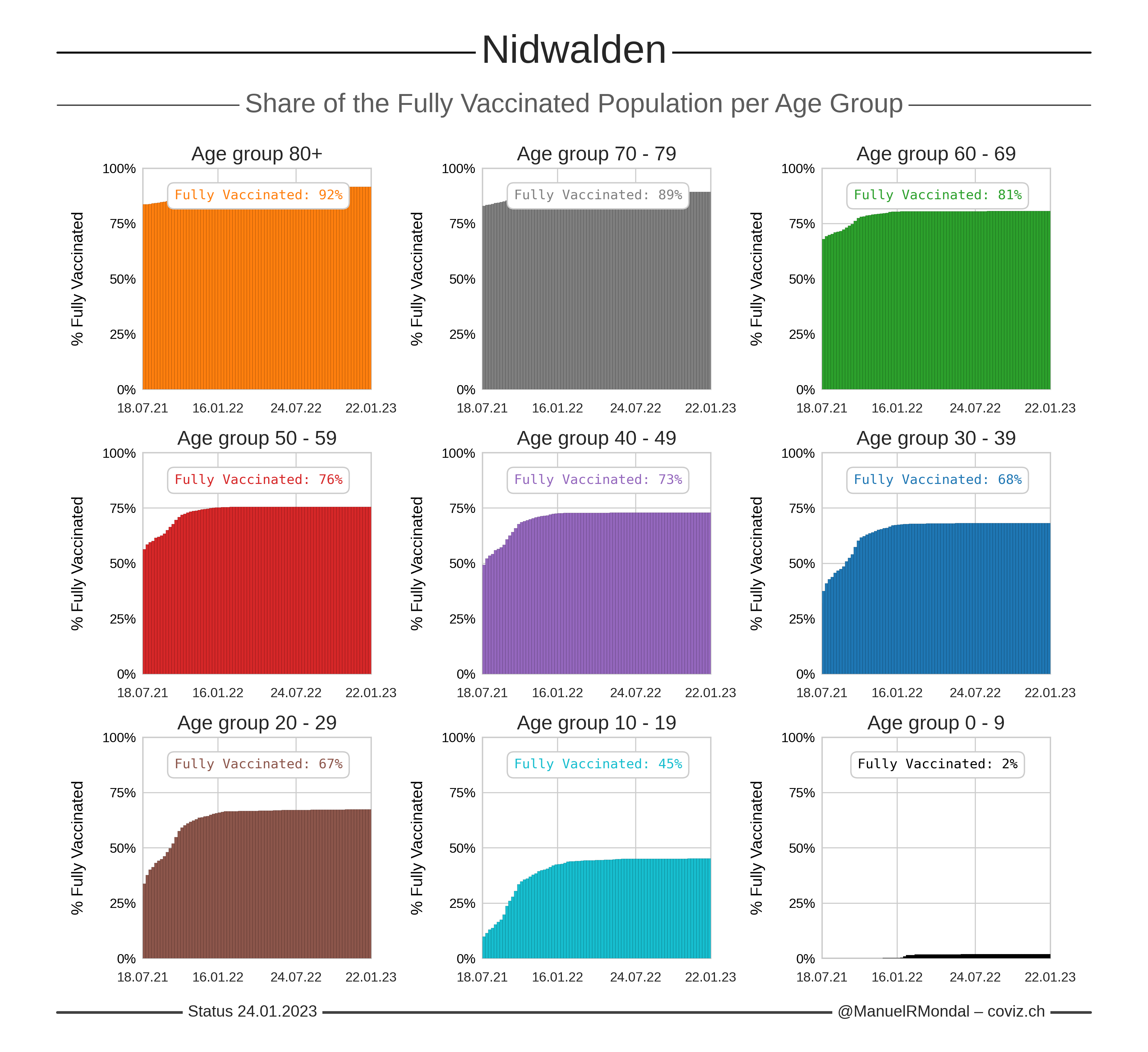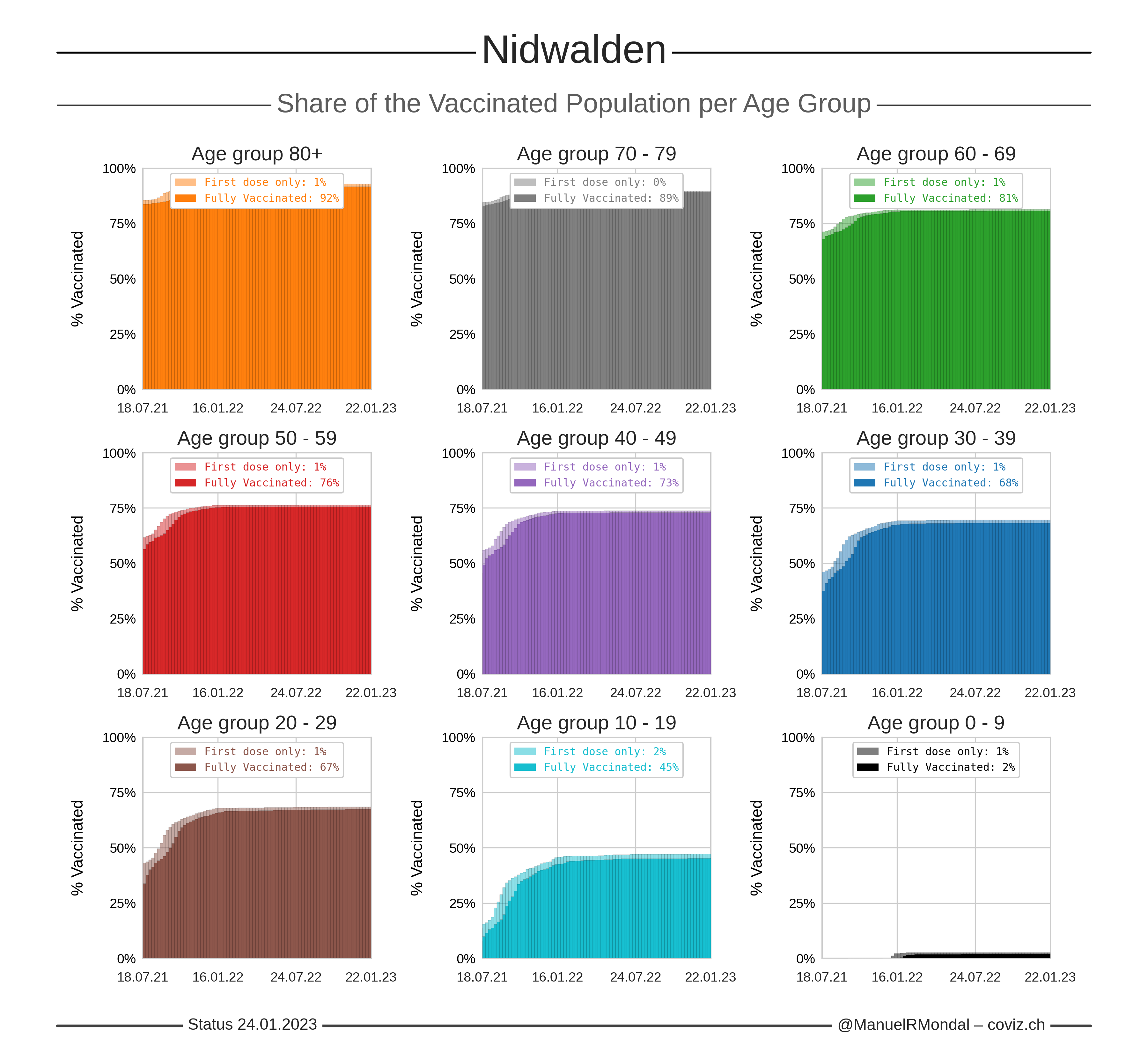Click the image to zoom.
Updated:
Number of first doses calculated from the total number of administered doses and assuming two doses for full vaccinations.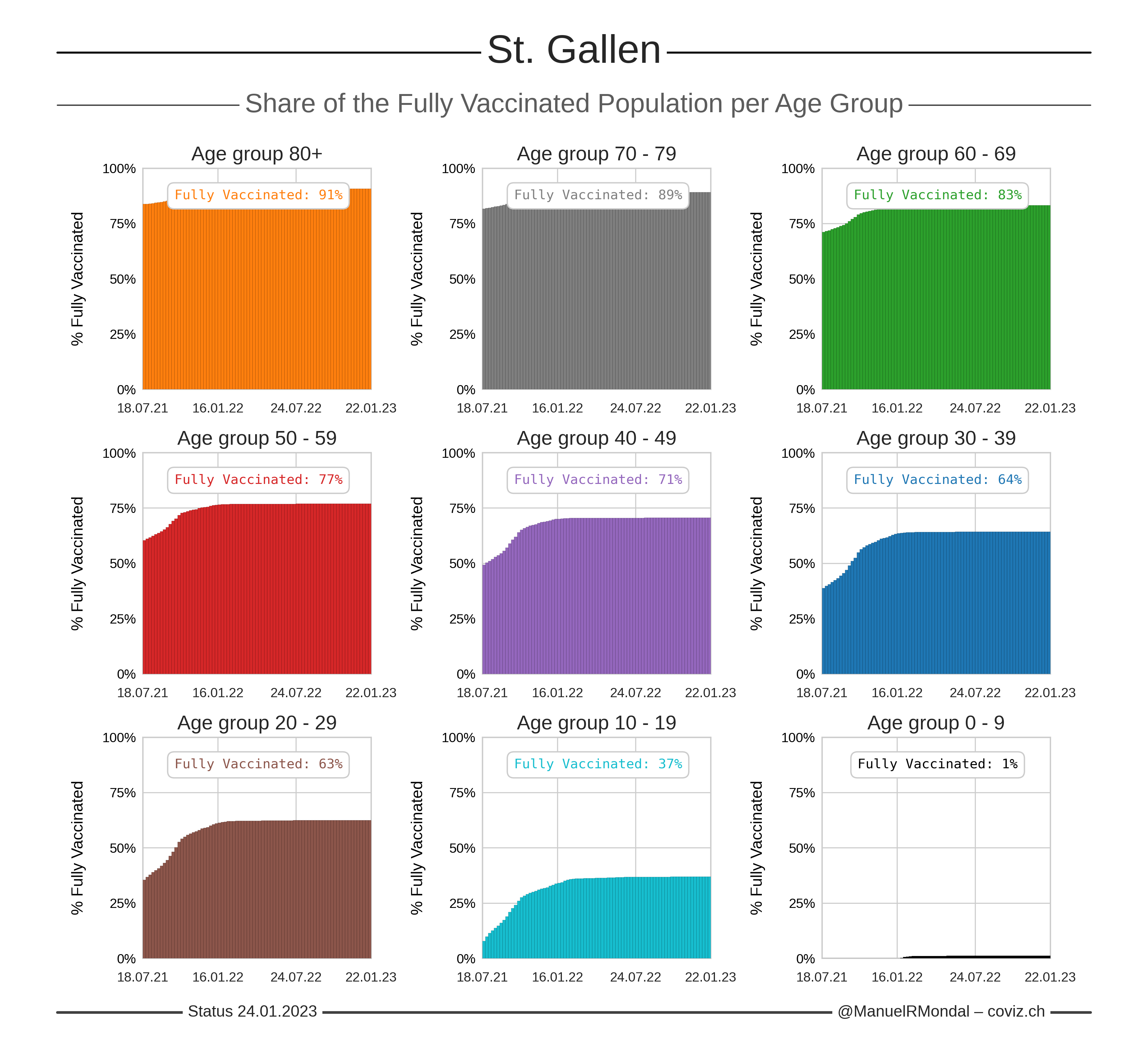Click the image to zoom.
Updated:
Number of first doses calculated from the total number of administered doses and assuming two doses for full vaccinations.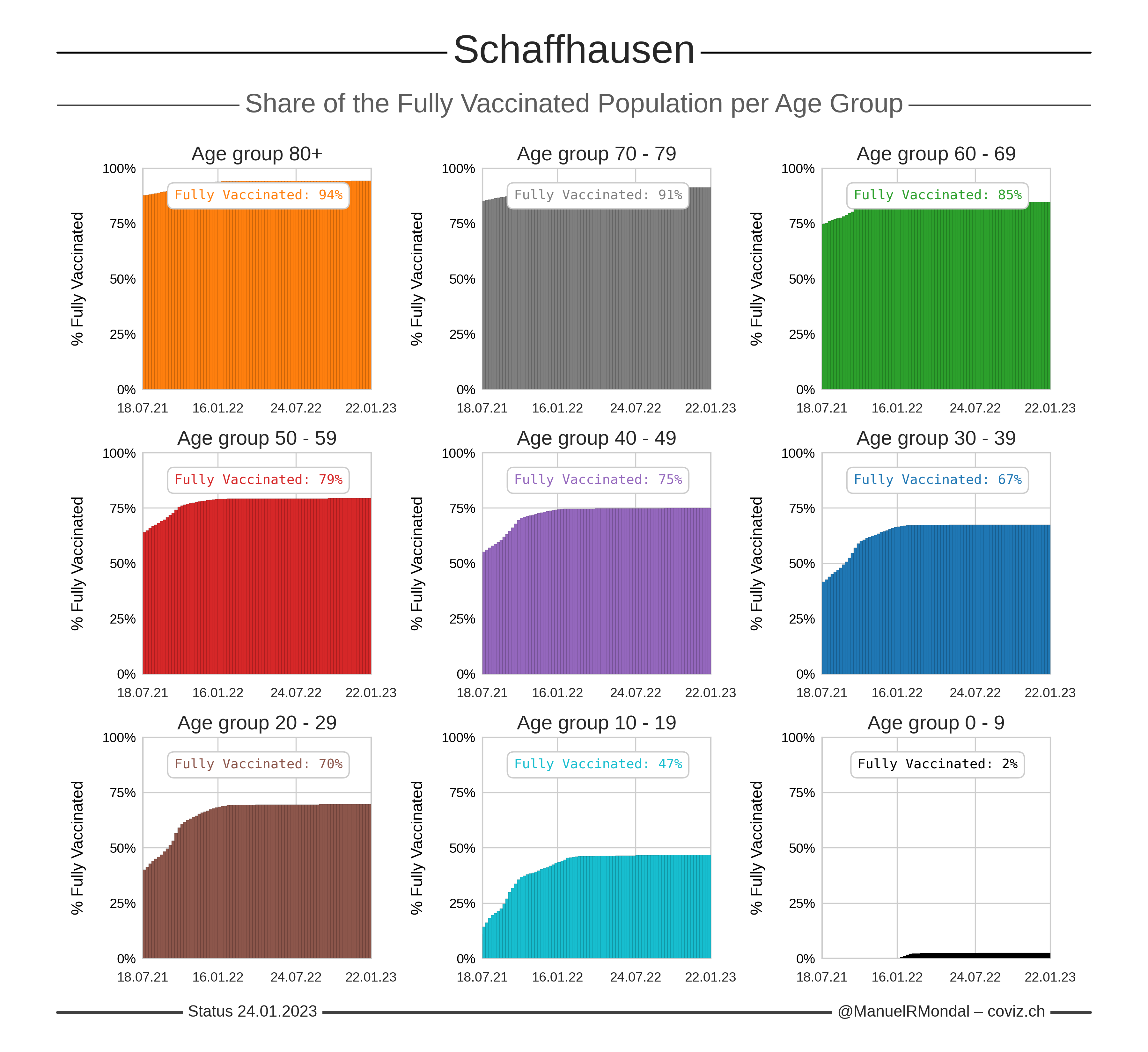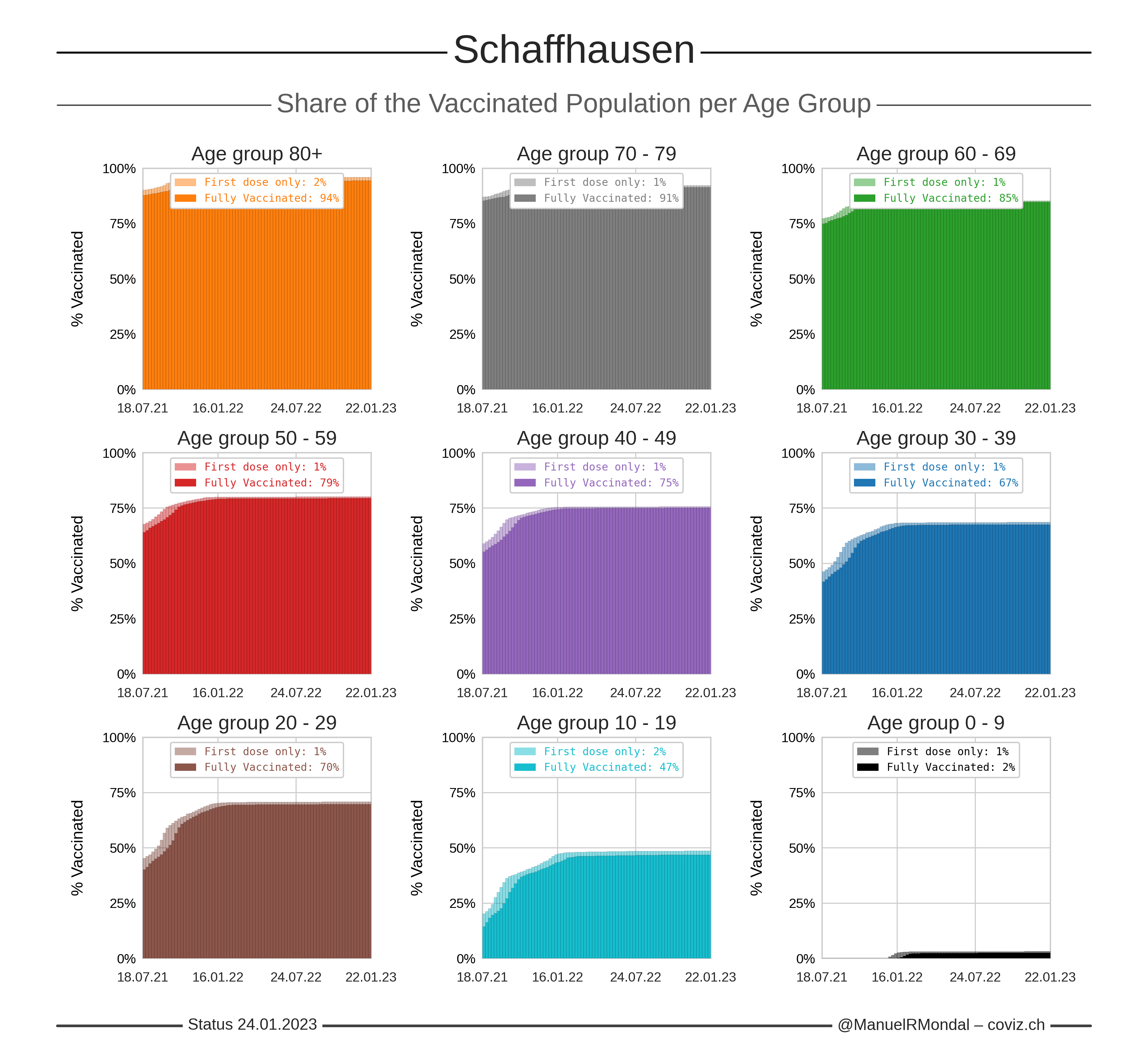Click the image to zoom.
Updated:
Number of first doses calculated from the total number of administered doses and assuming two doses for full vaccinations.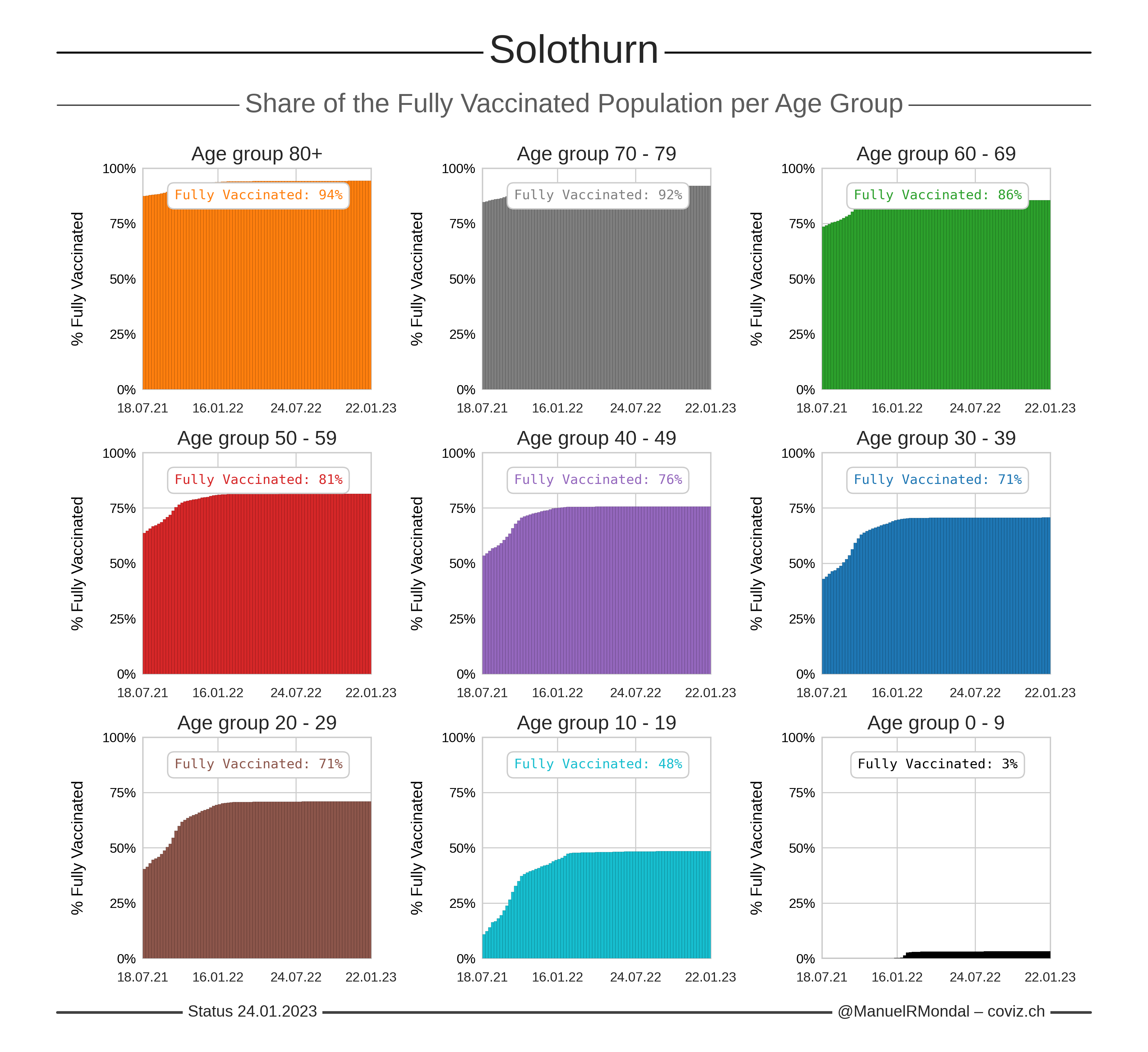Click the image to zoom.
Updated:
Number of first doses calculated from the total number of administered doses and assuming two doses for full vaccinations.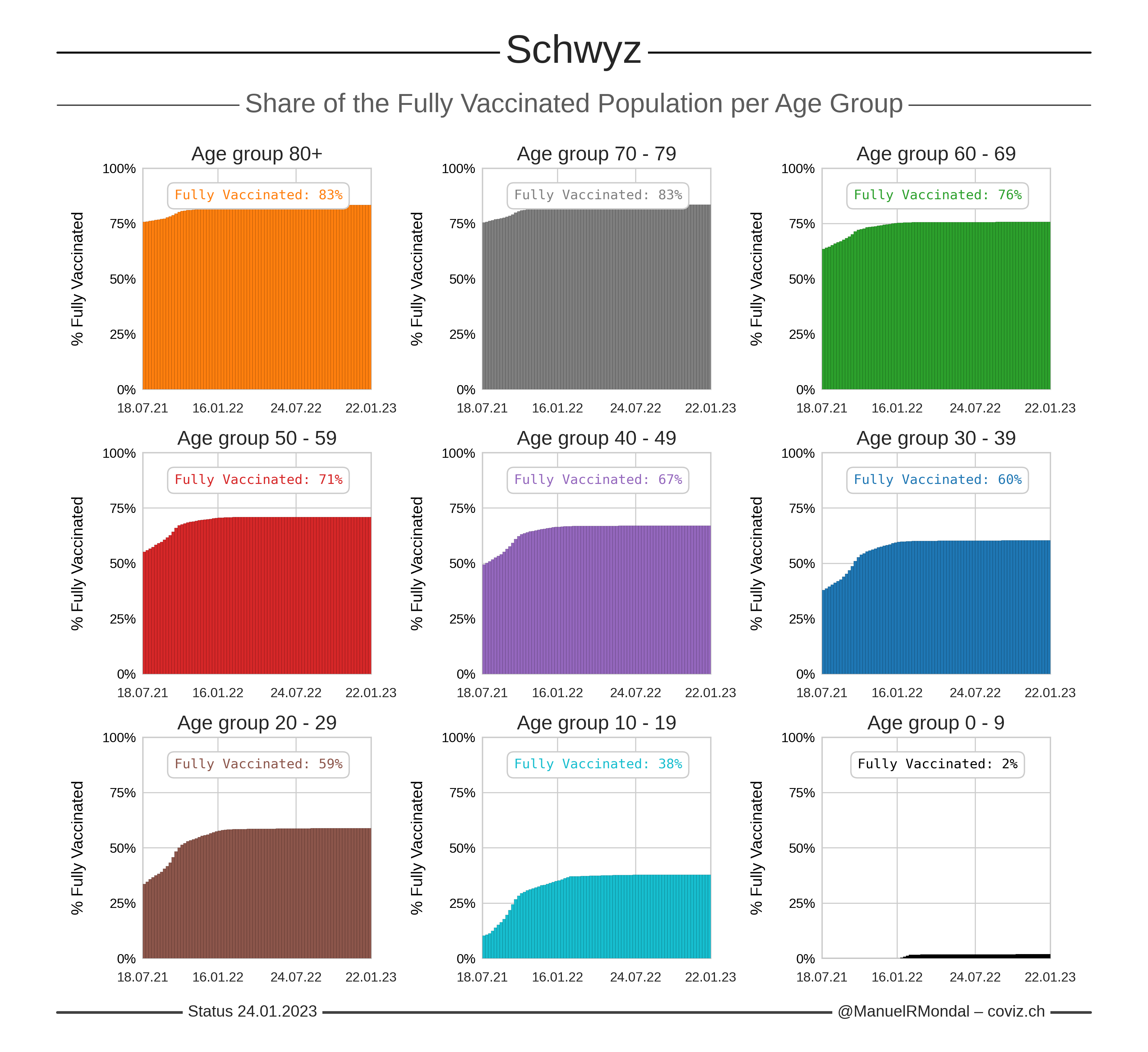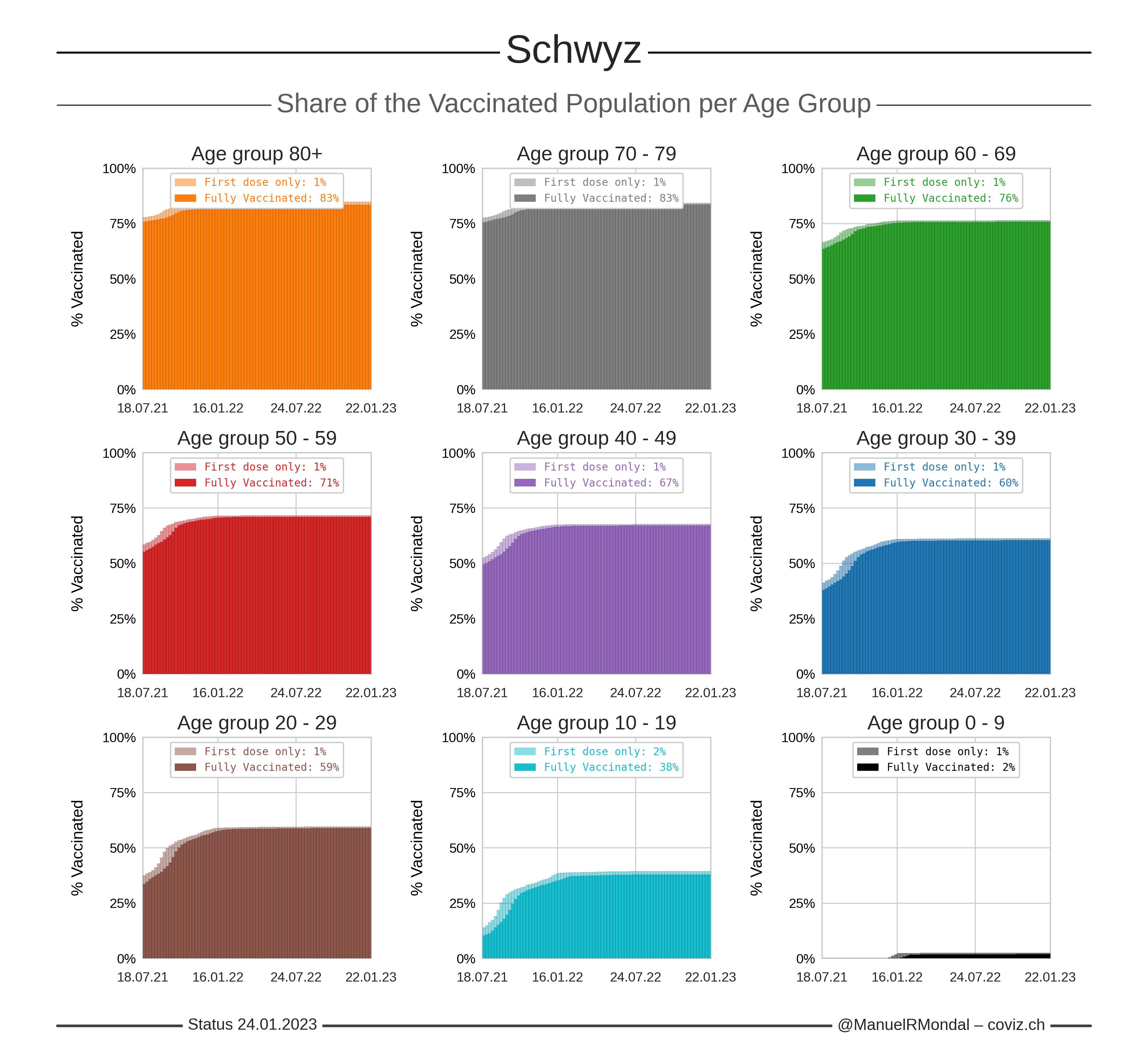Click the image to zoom.
Updated:
Number of first doses calculated from the total number of administered doses and assuming two doses for full vaccinations.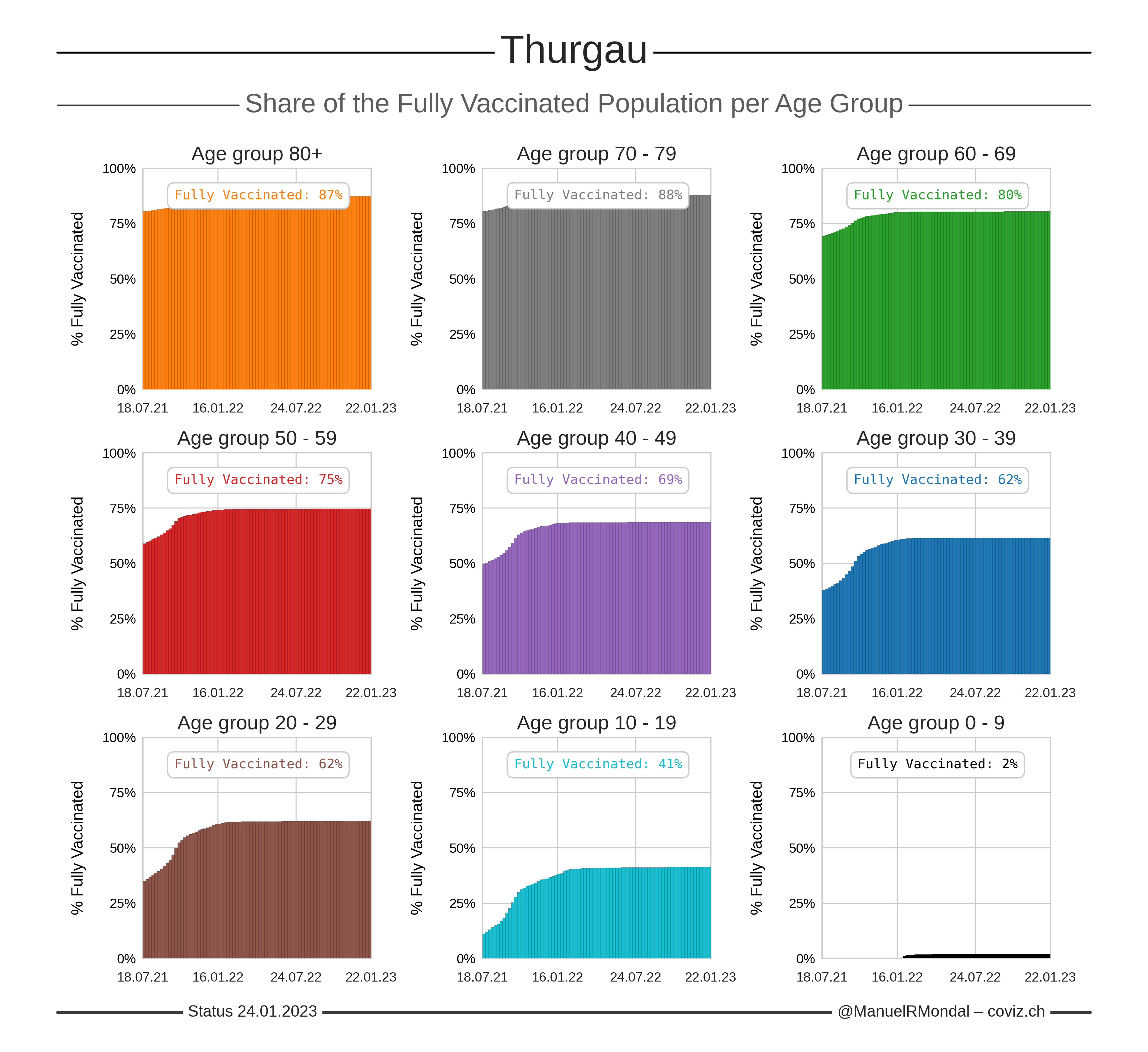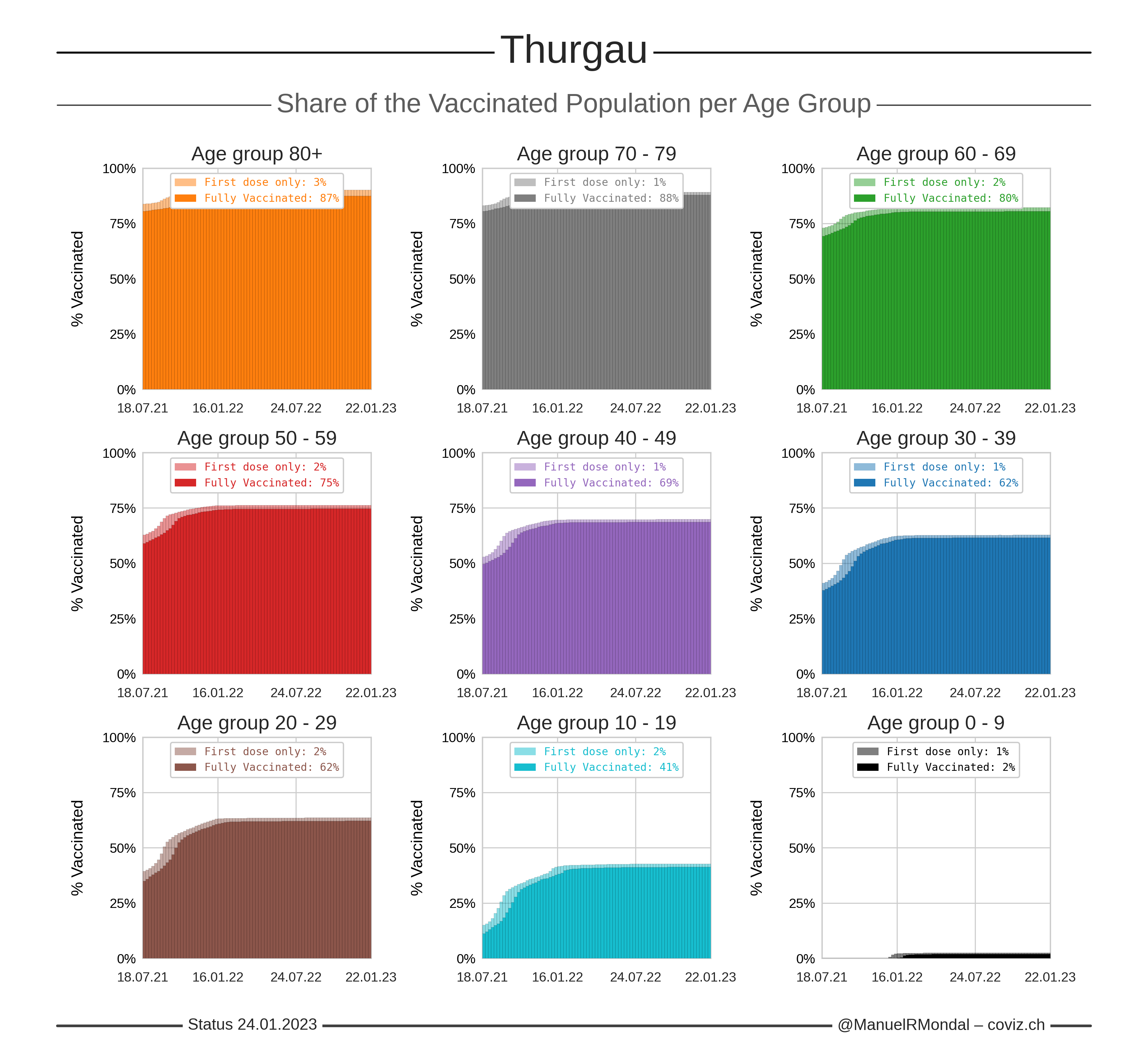Click the image to zoom.
Updated:
Number of first doses calculated from the total number of administered doses and assuming two doses for full vaccinations.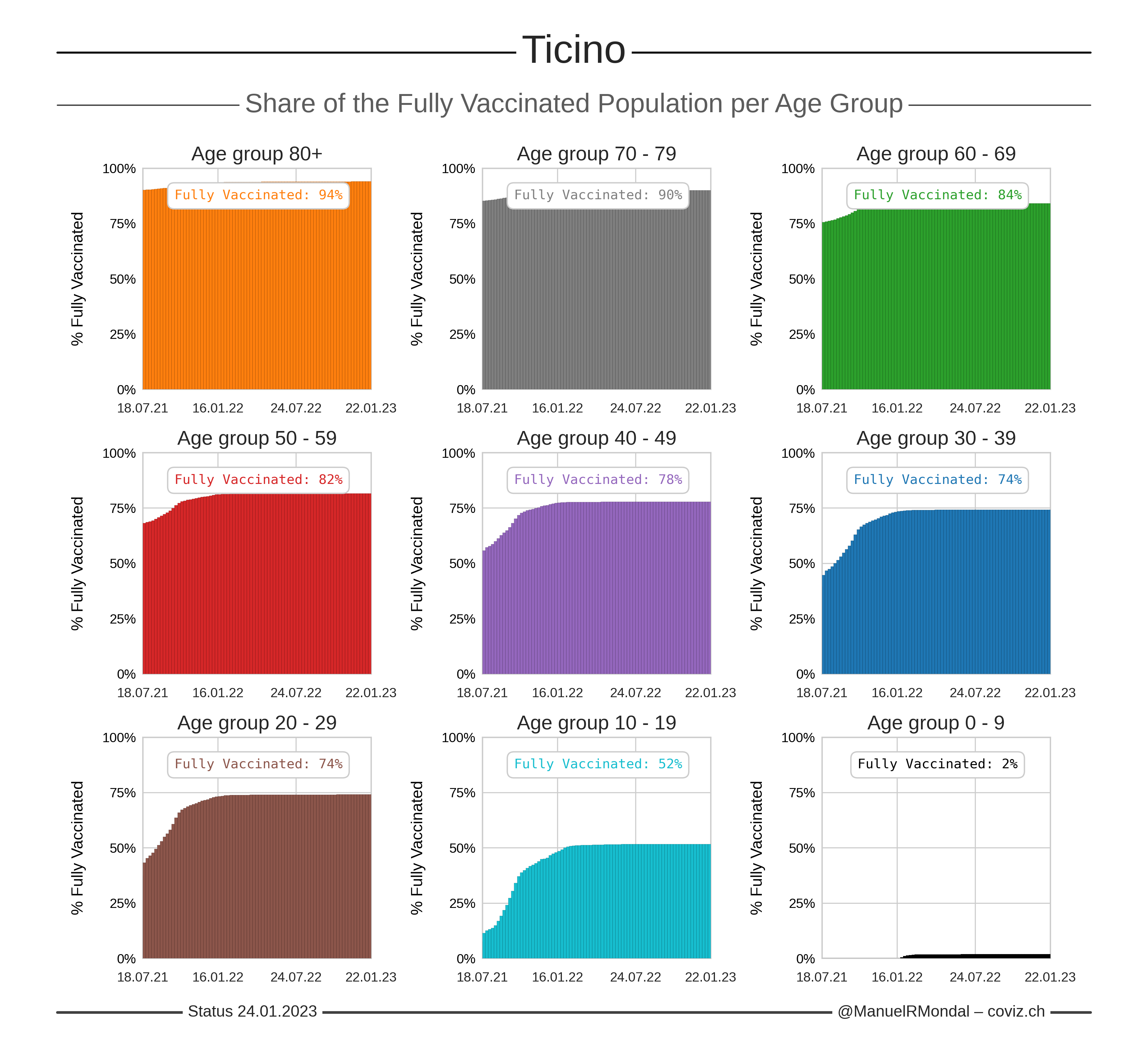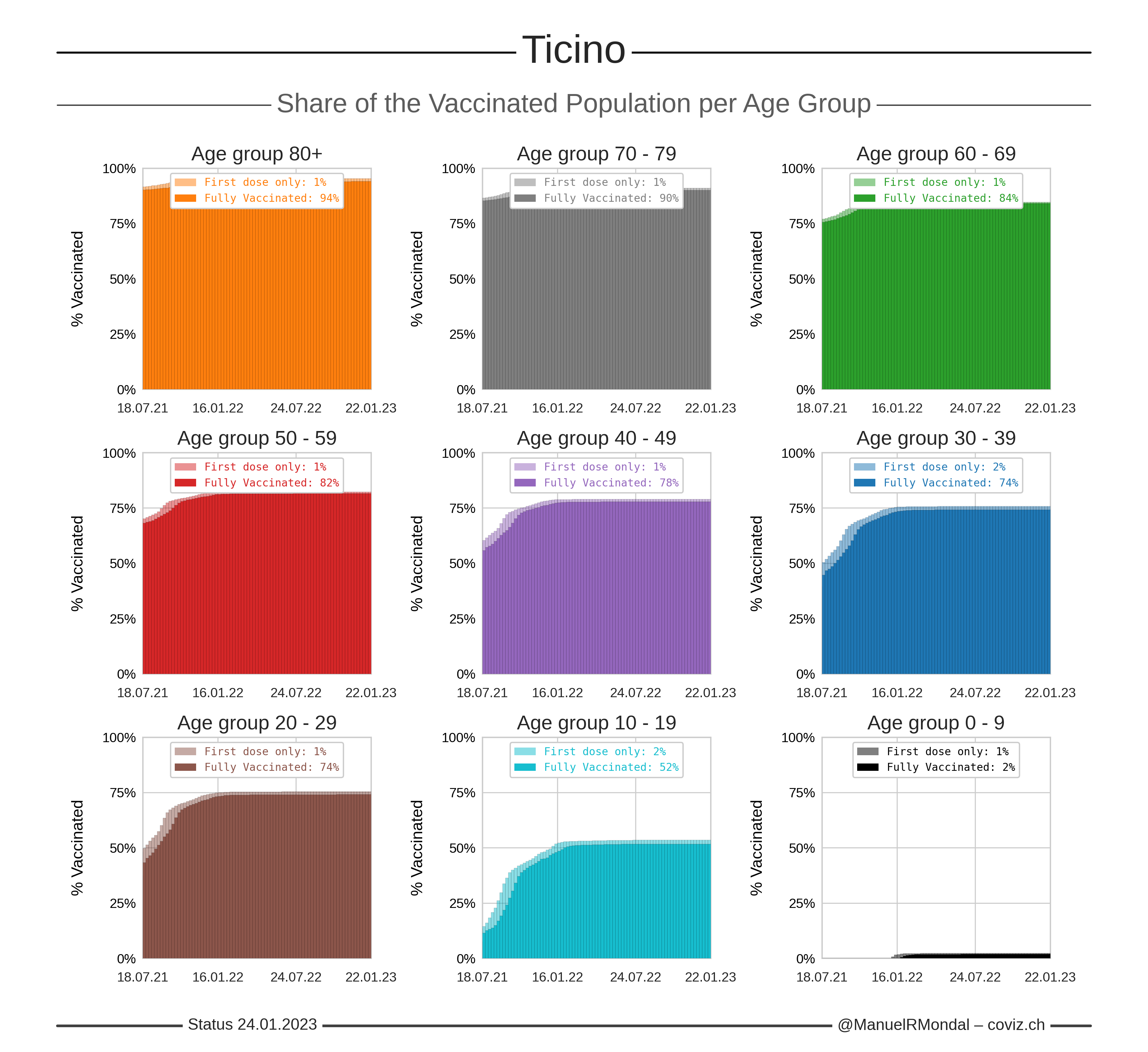Click the image to zoom.
Updated:
Number of first doses calculated from the total number of administered doses and assuming two doses for full vaccinations.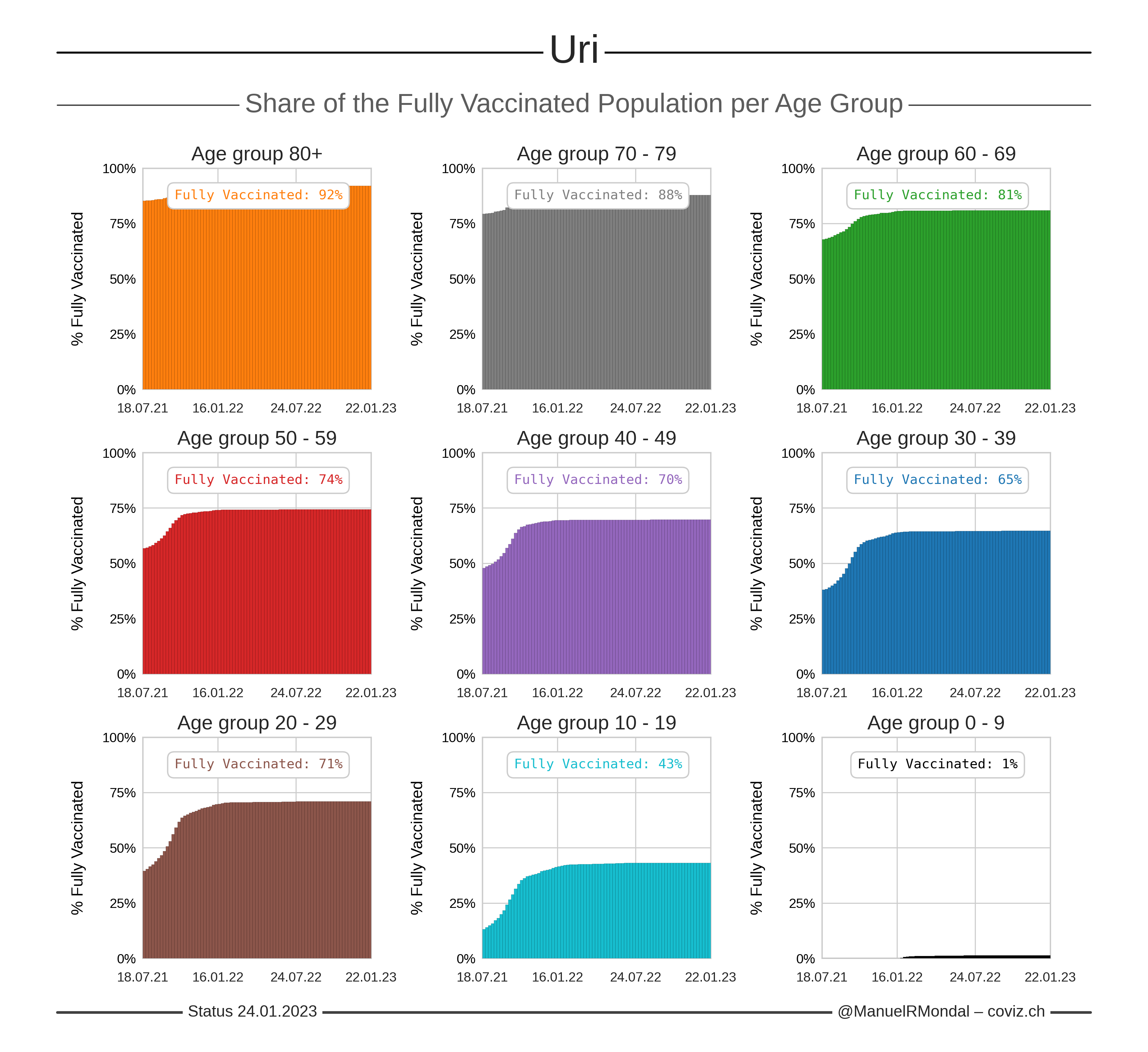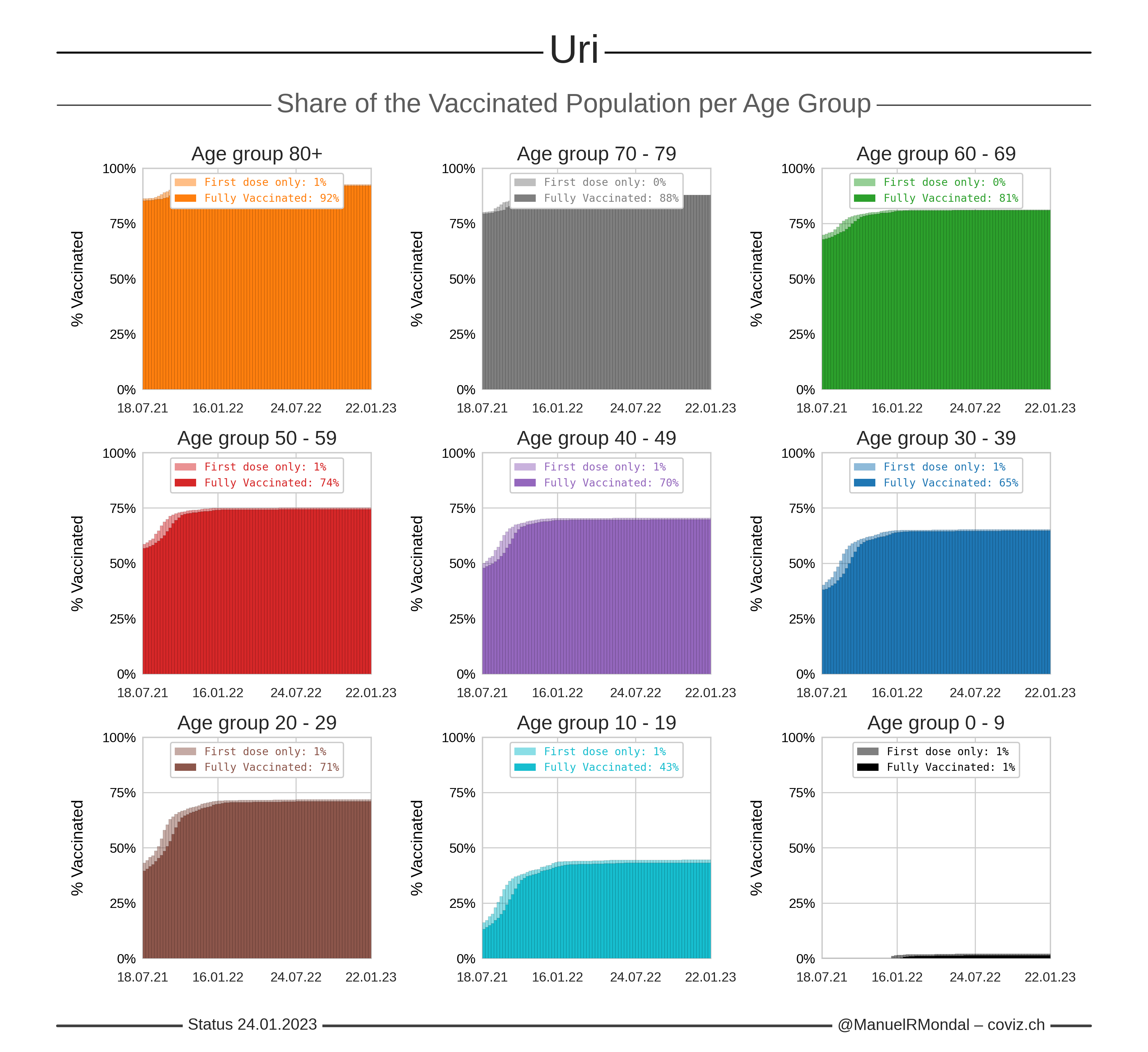Click the image to zoom.
Updated:
Number of first doses calculated from the total number of administered doses and assuming two doses for full vaccinations.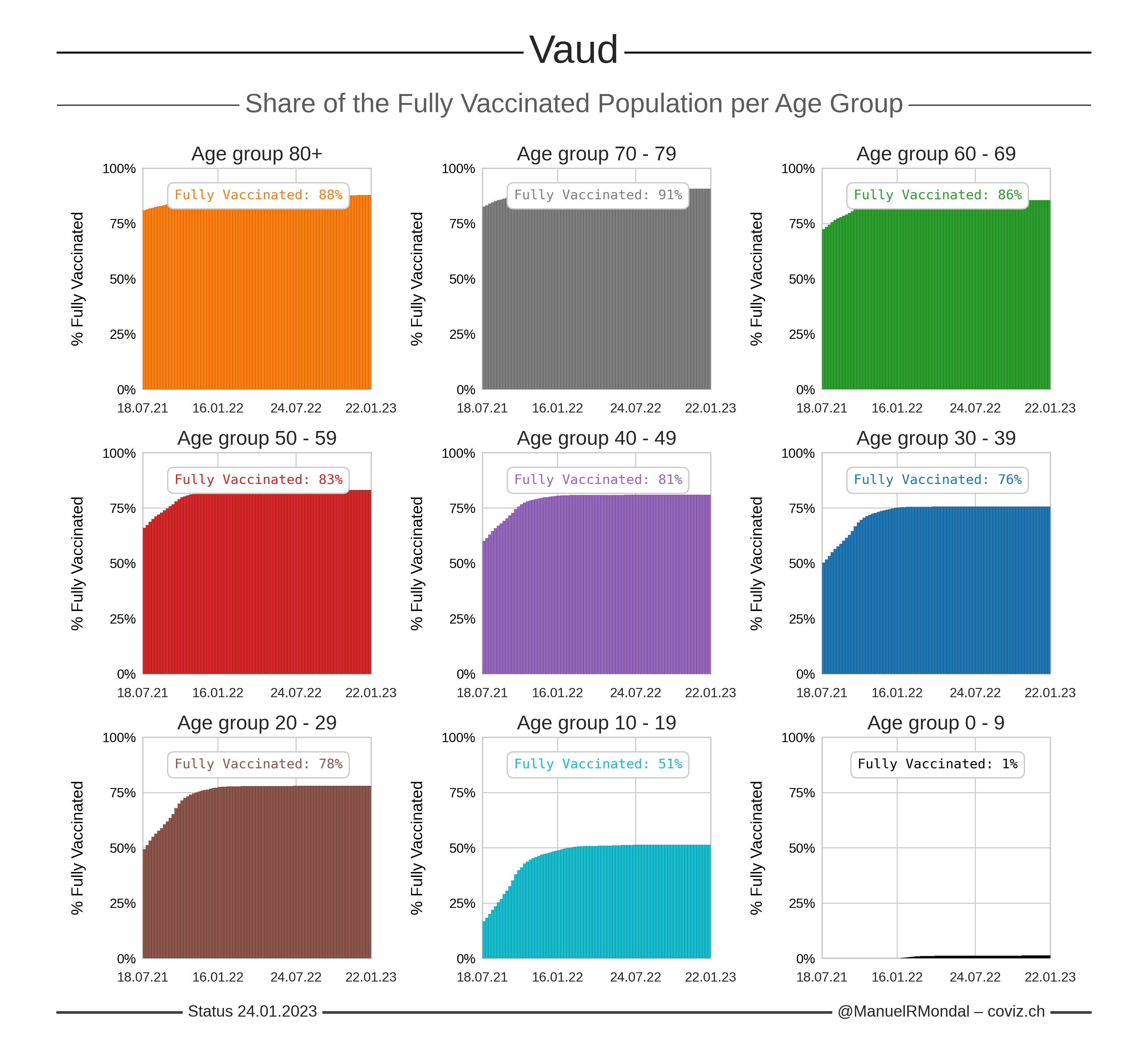Click the image to zoom.
Updated:
Number of first doses calculated from the total number of administered doses and assuming two doses for full vaccinations.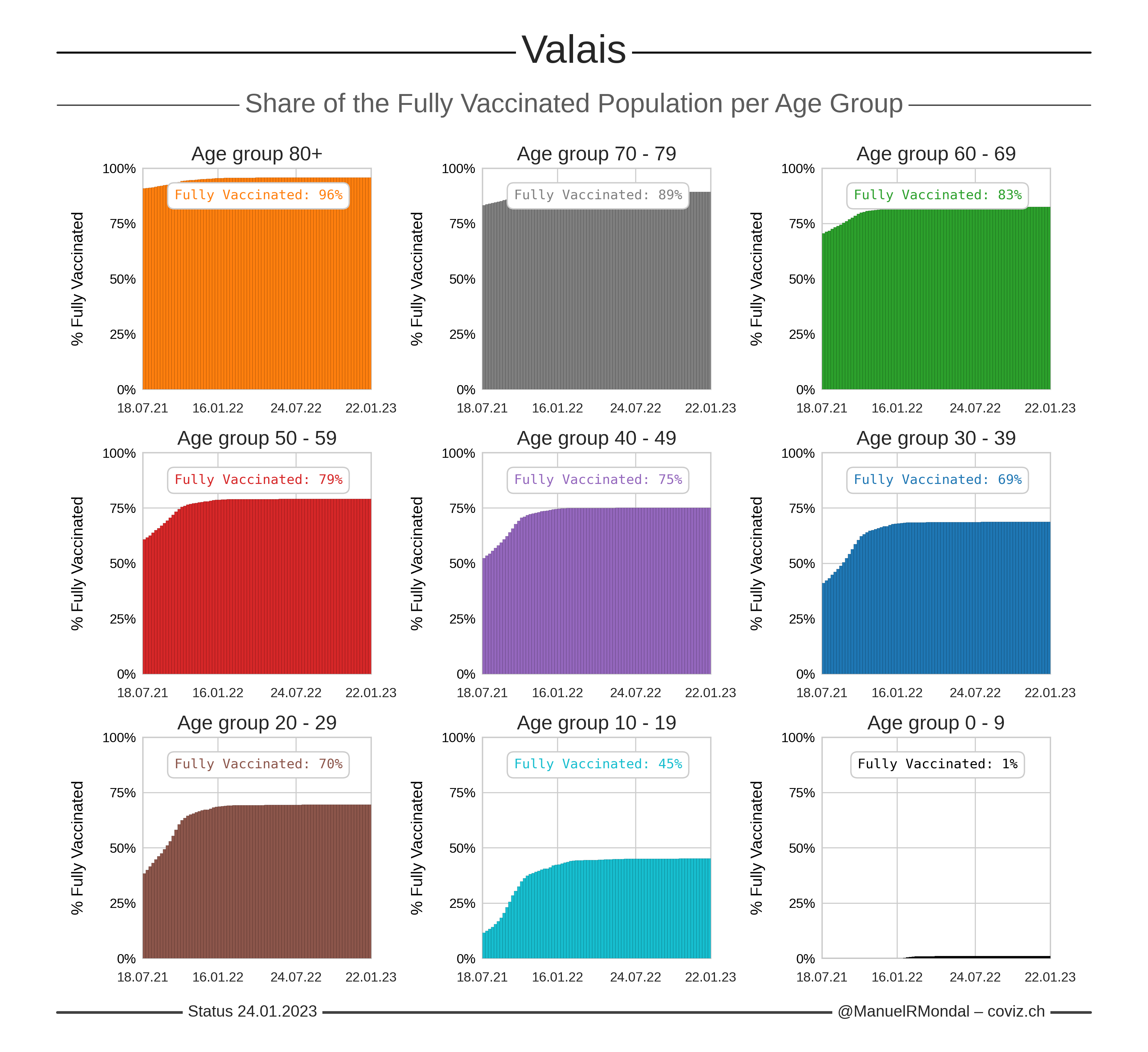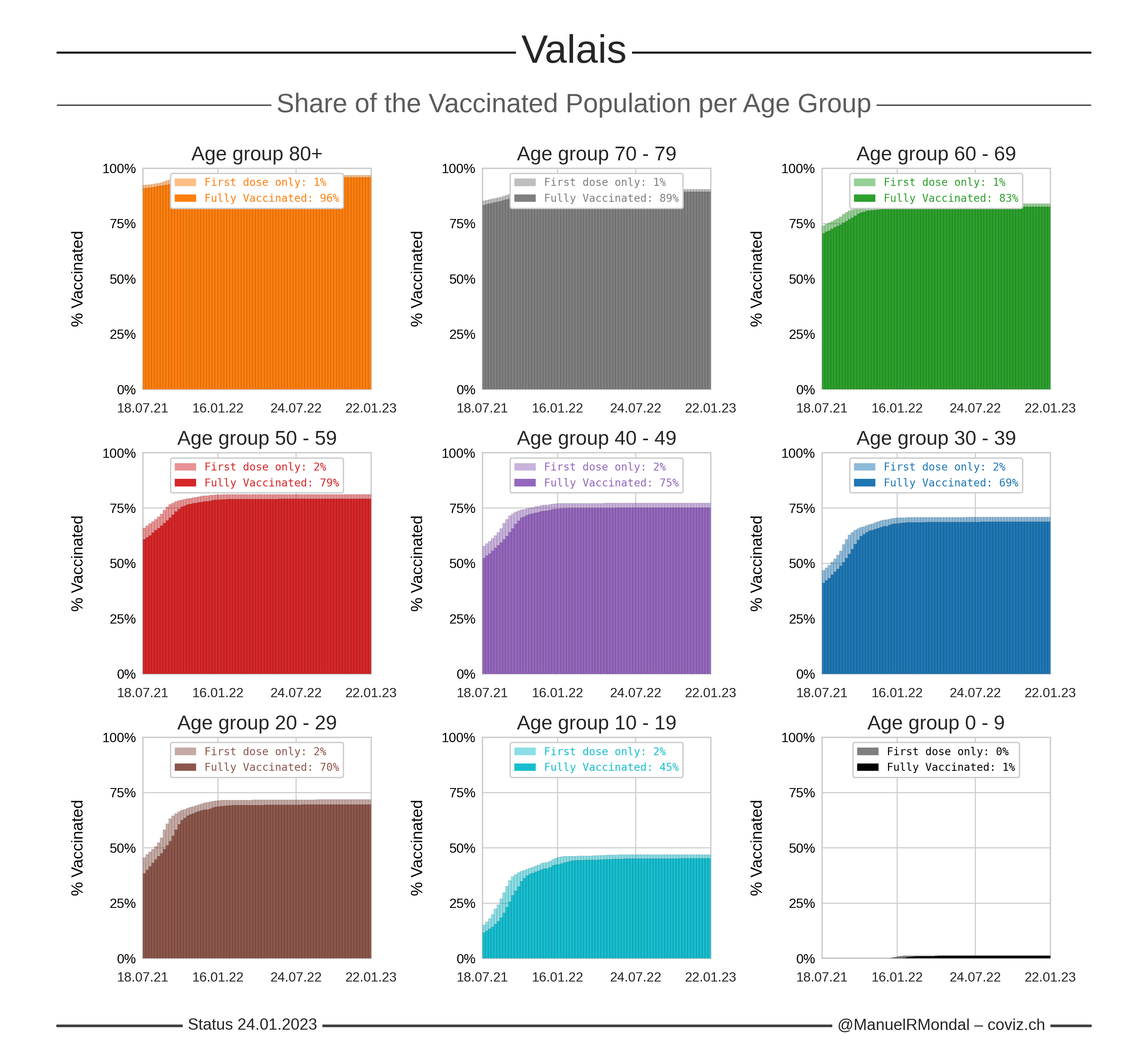Click the image to zoom.
Updated:
Number of first doses calculated from the total number of administered doses and assuming two doses for full vaccinations.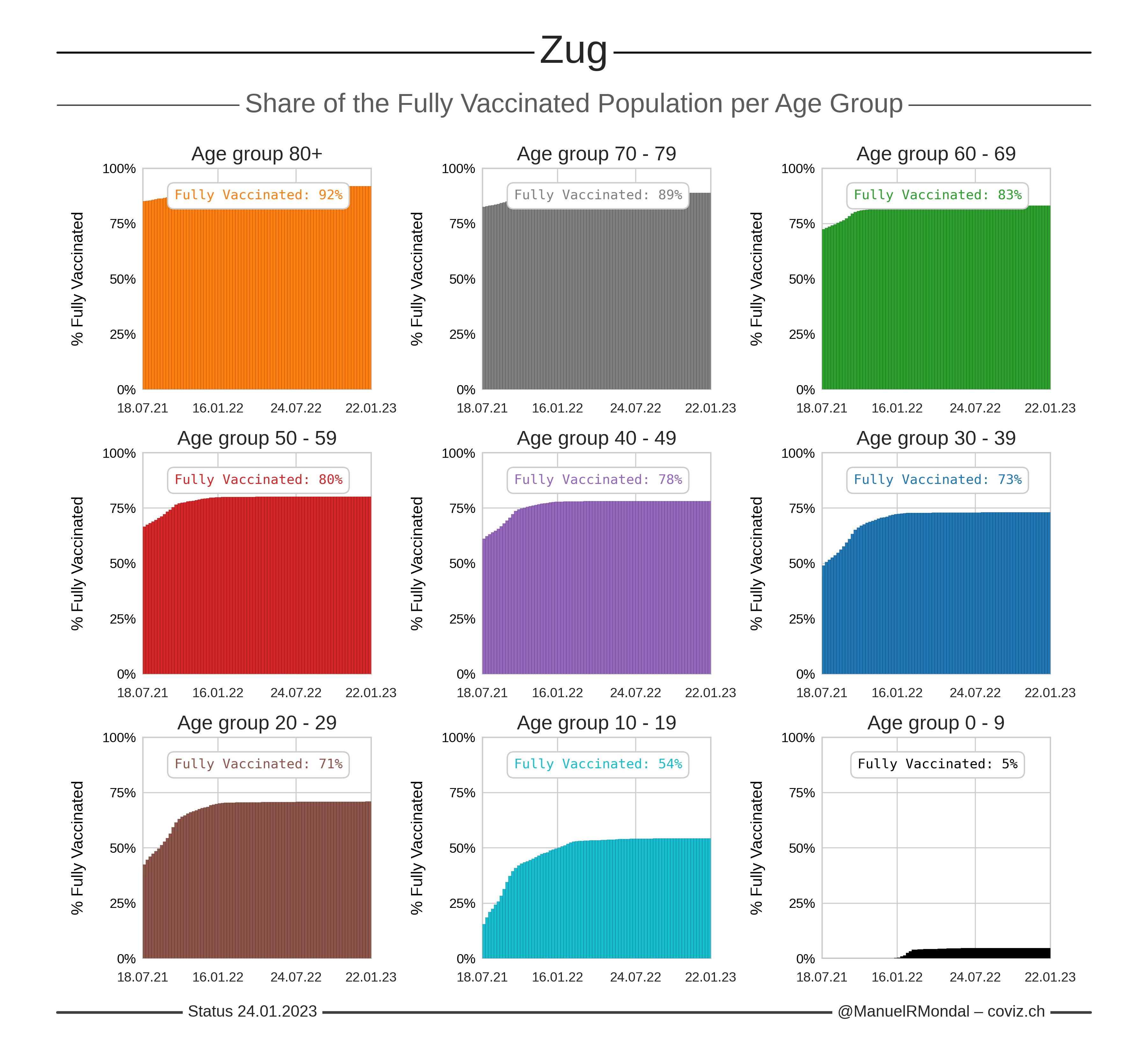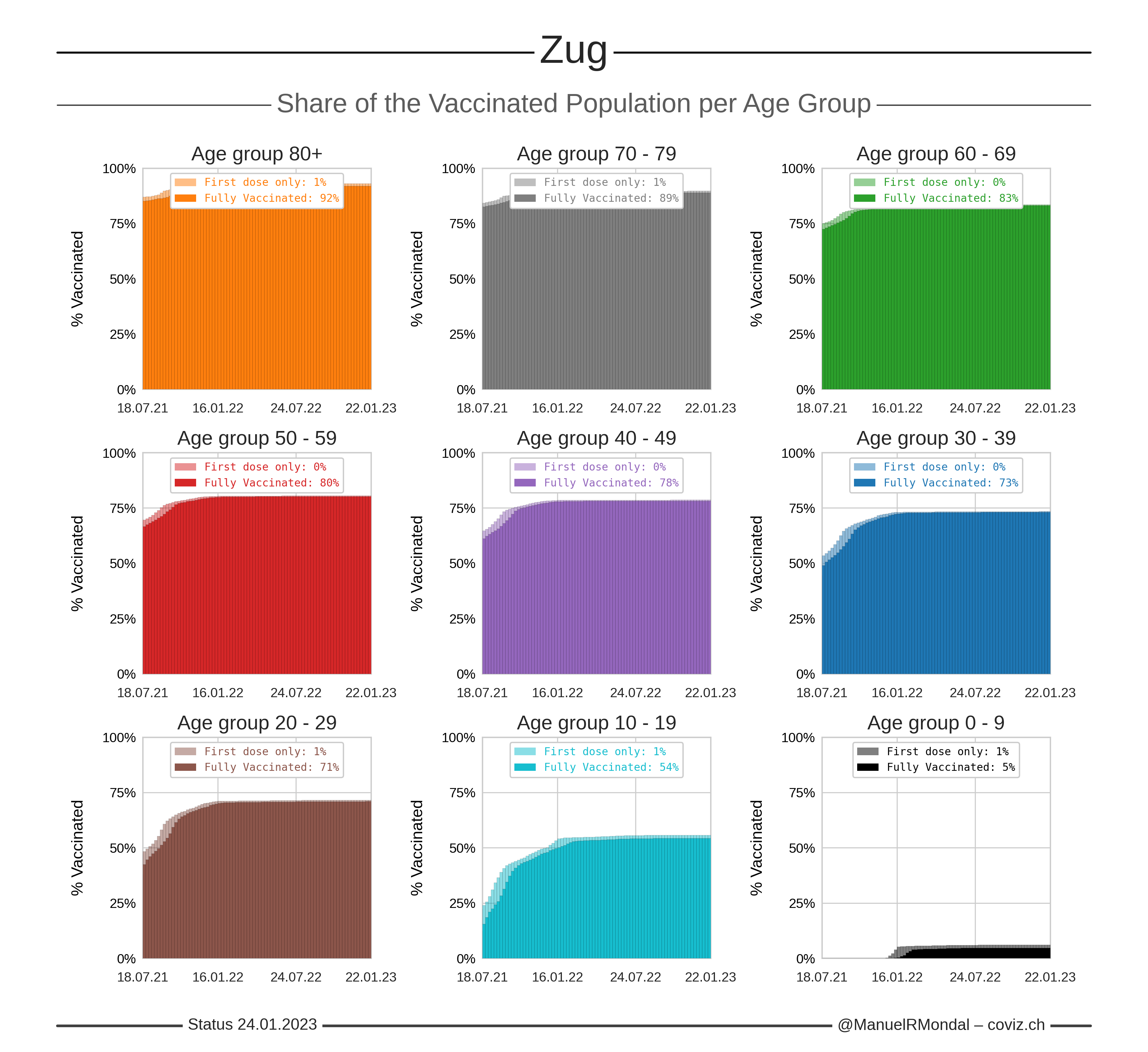Click the image to zoom.